# NCERT Solutions for Class 6 Maths Chapter 7 – Fractions

NCERT Solutions for Class 6 Maths Chapter 7 – Fractions comprises of the 6 Exercises

### NCERT Solutions for Class 6 Maths Chapter 7 Exercise 7.1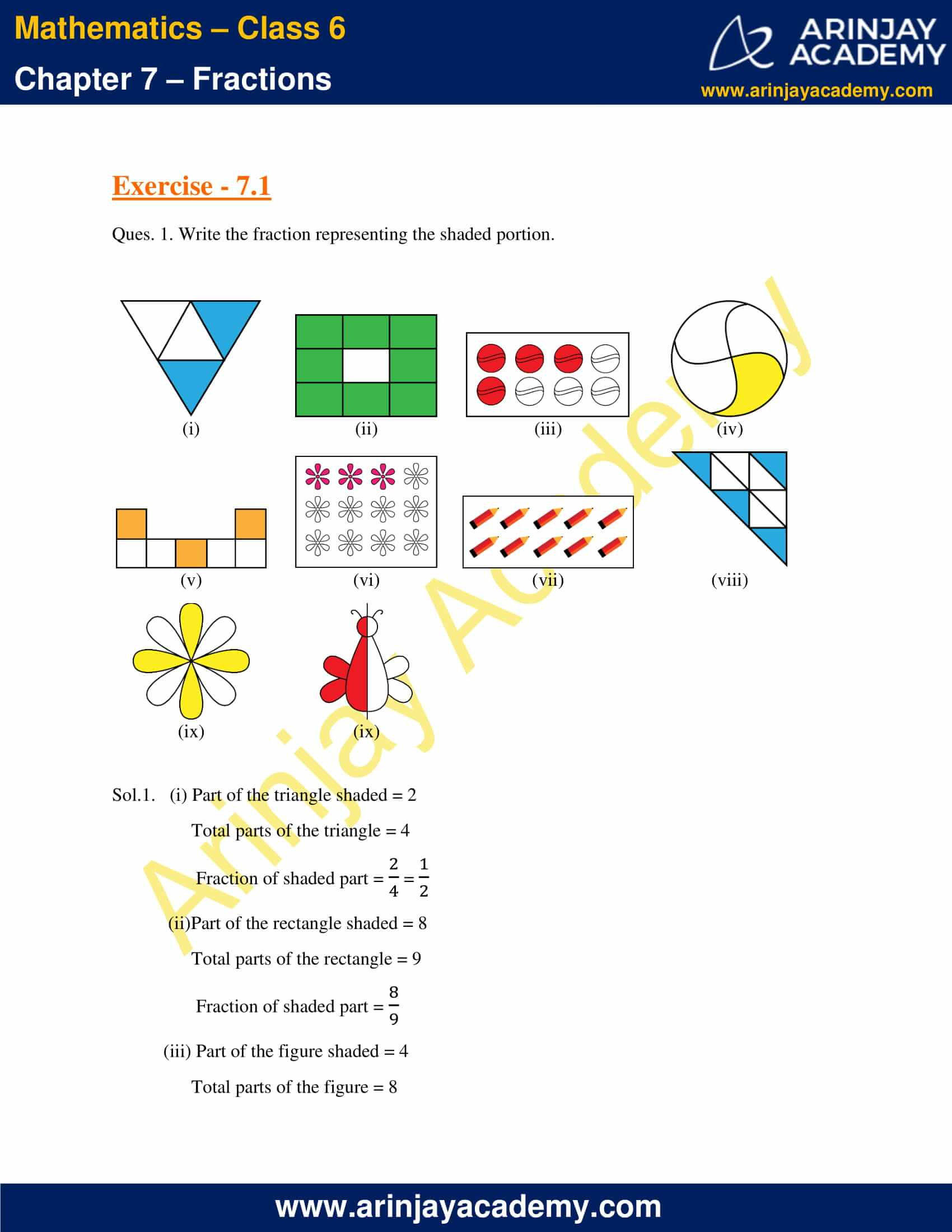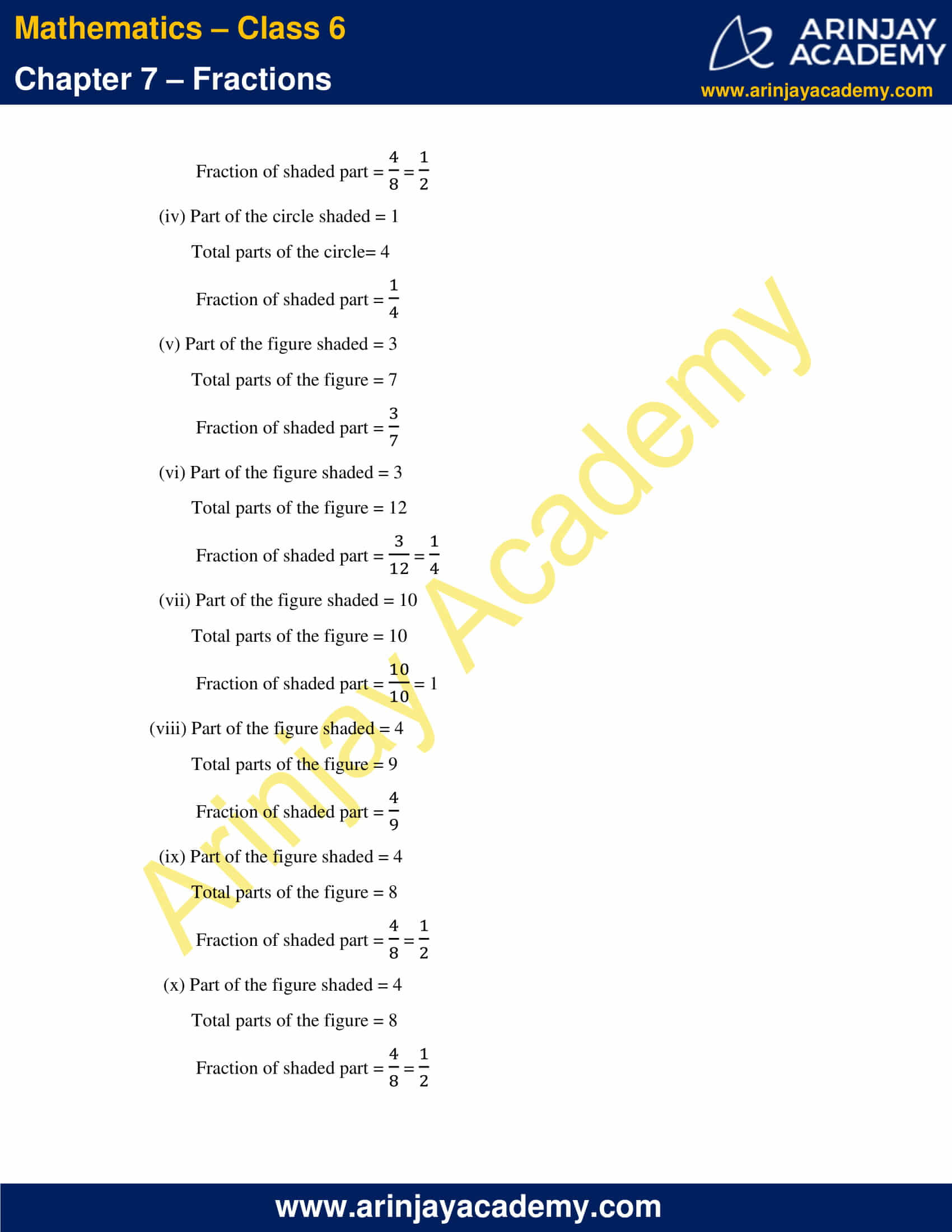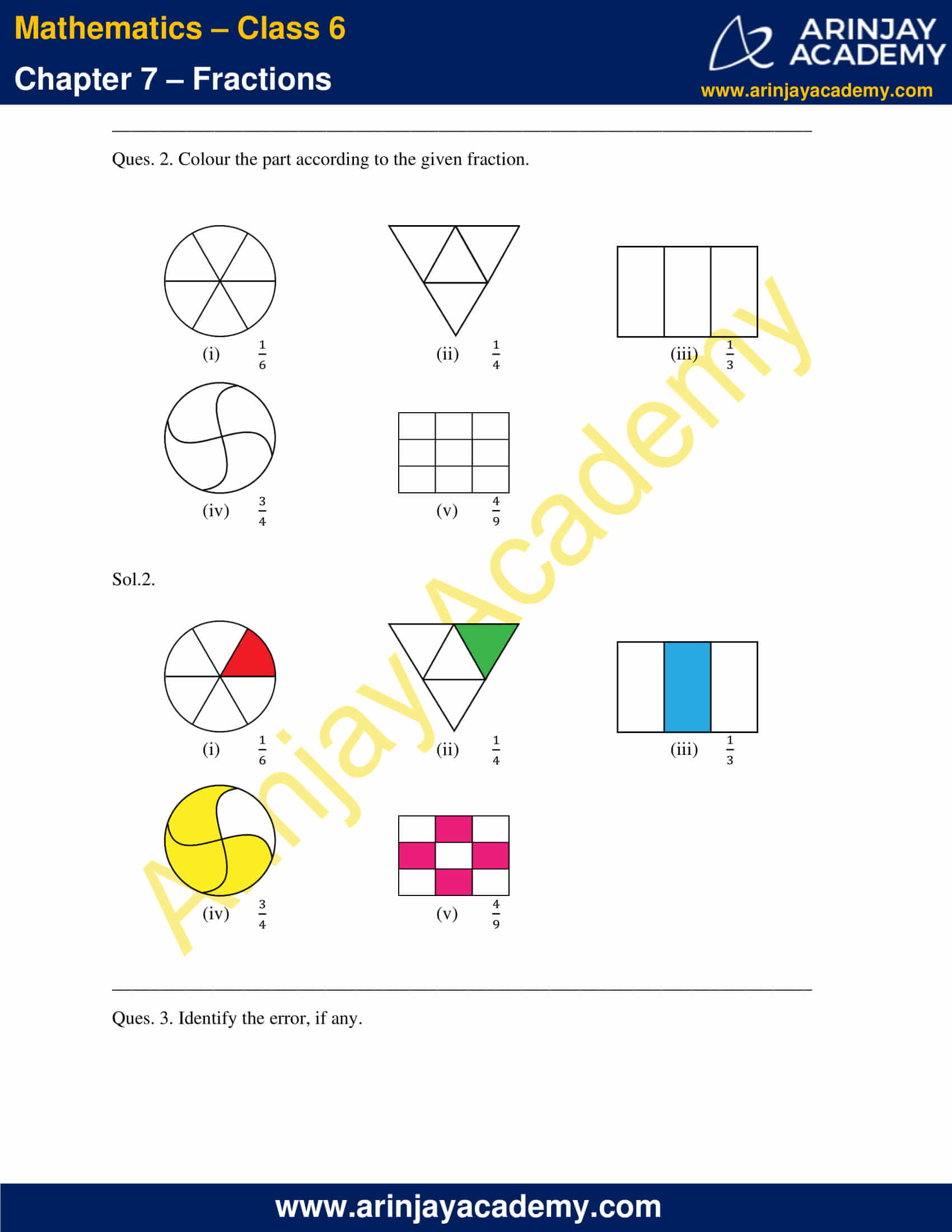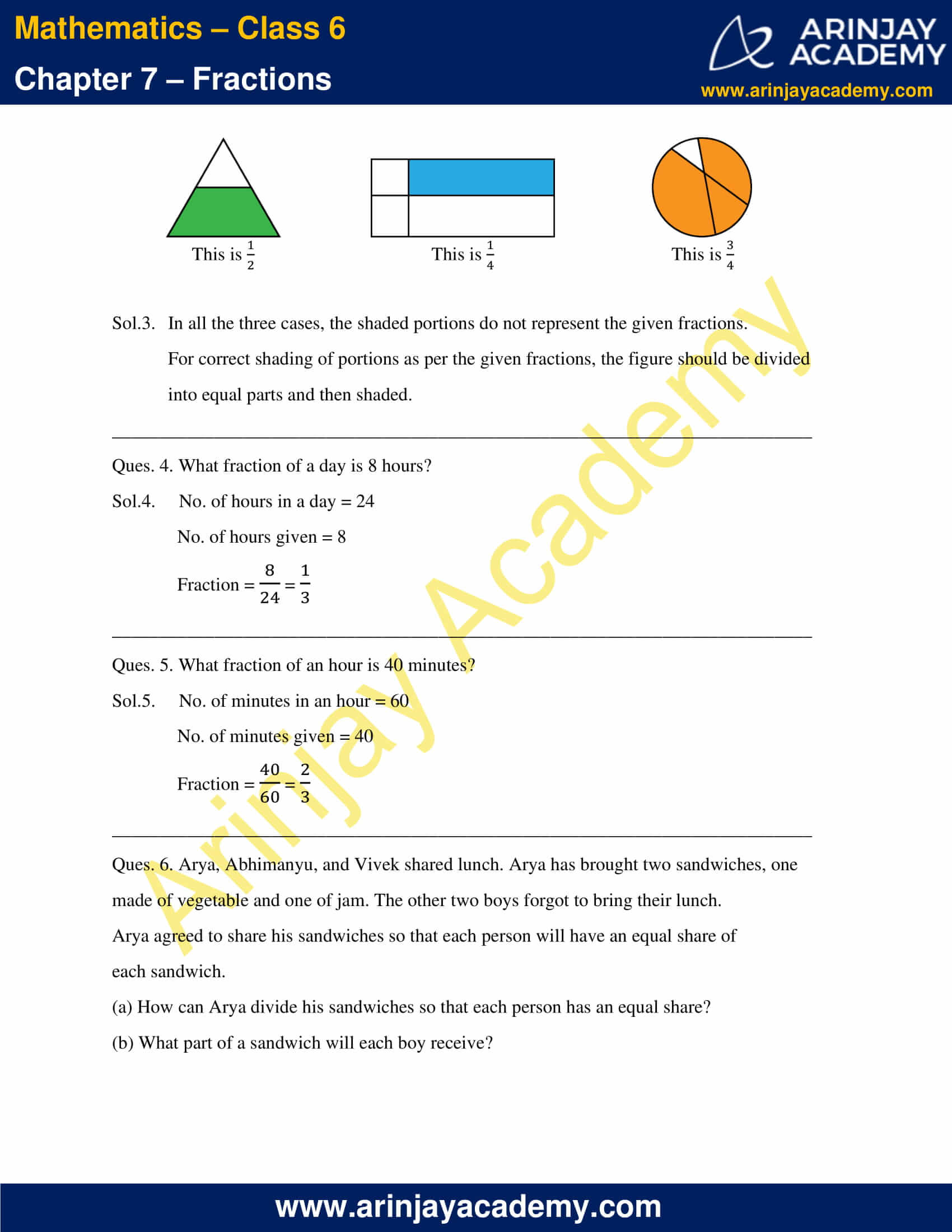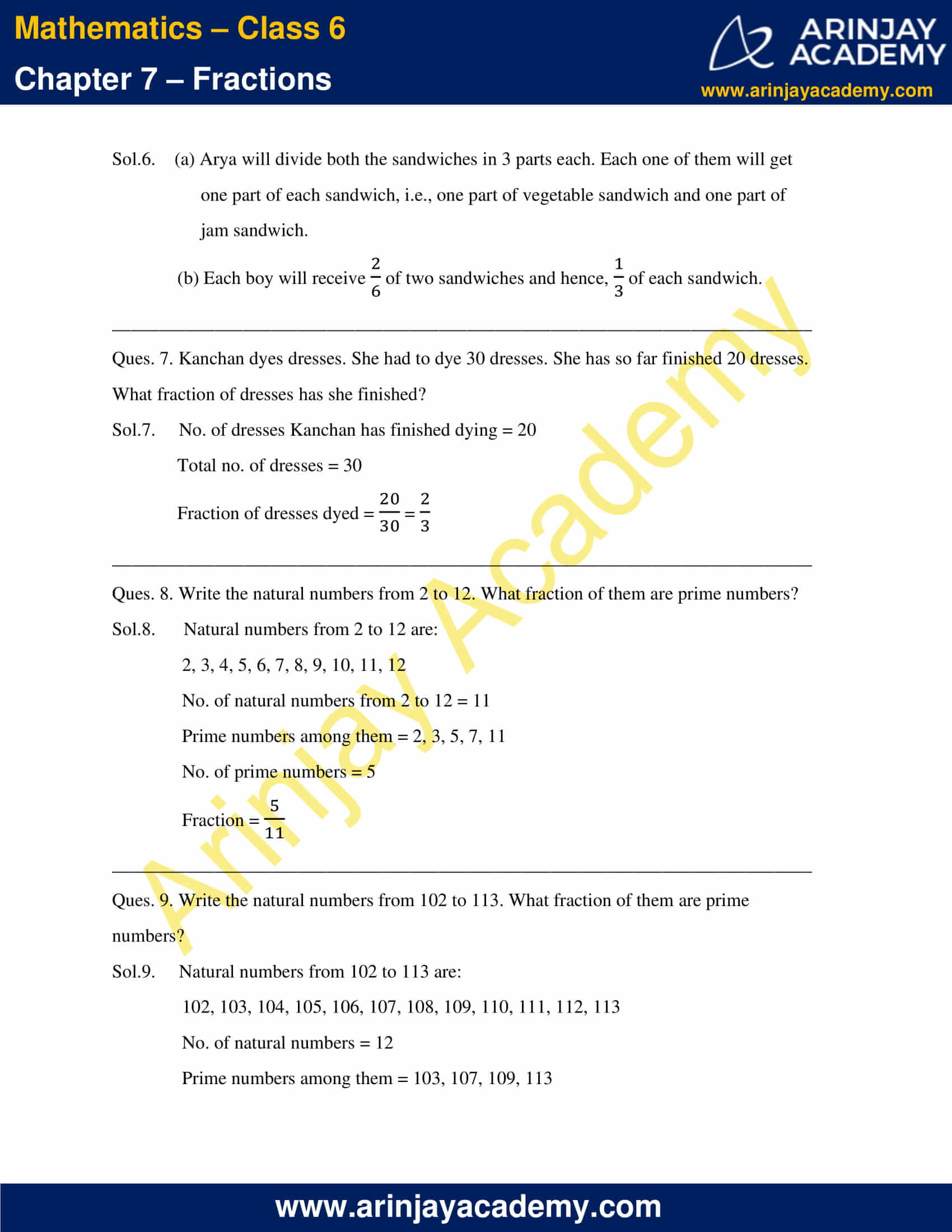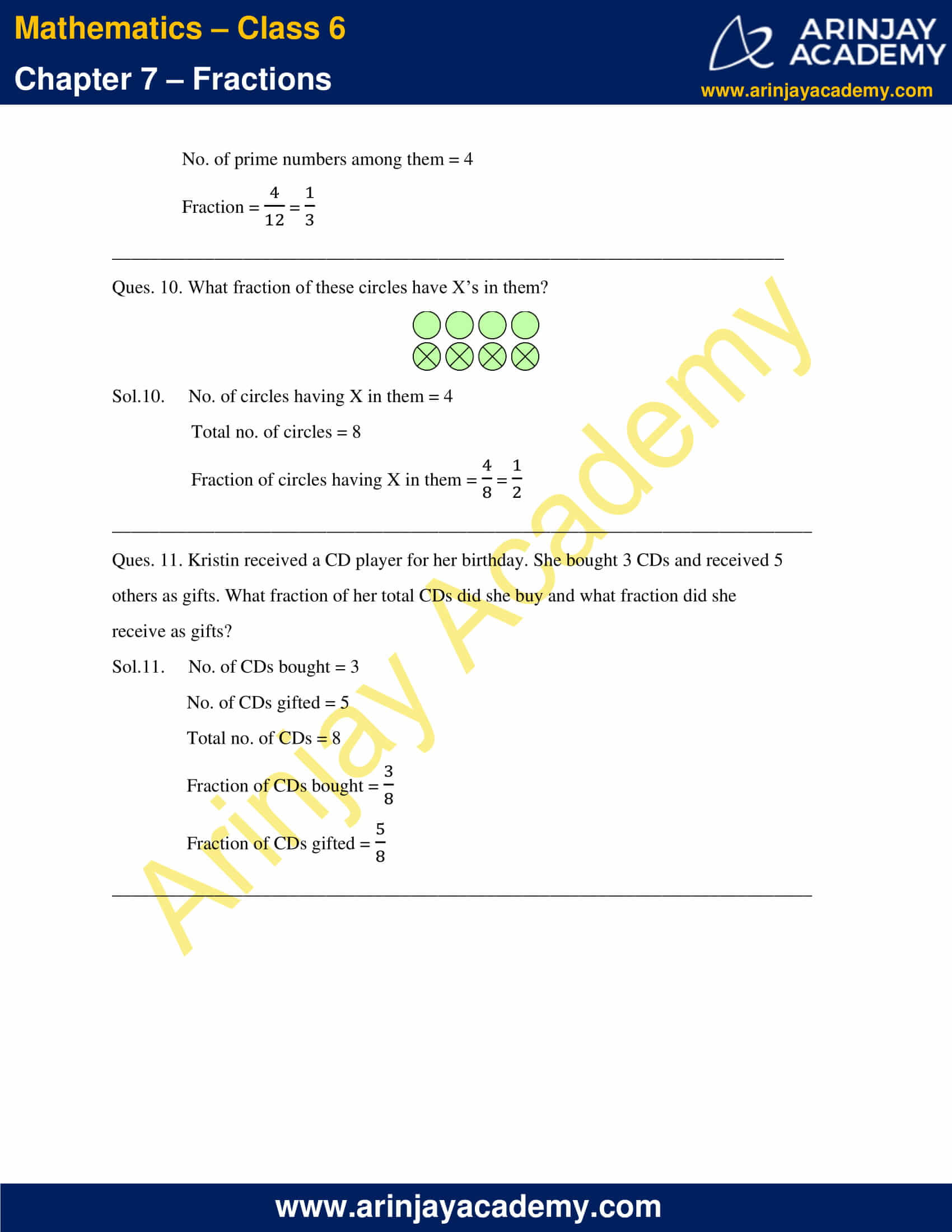### NCERT Solutions for Class 6 Maths Chapter 7 Exercise 7.2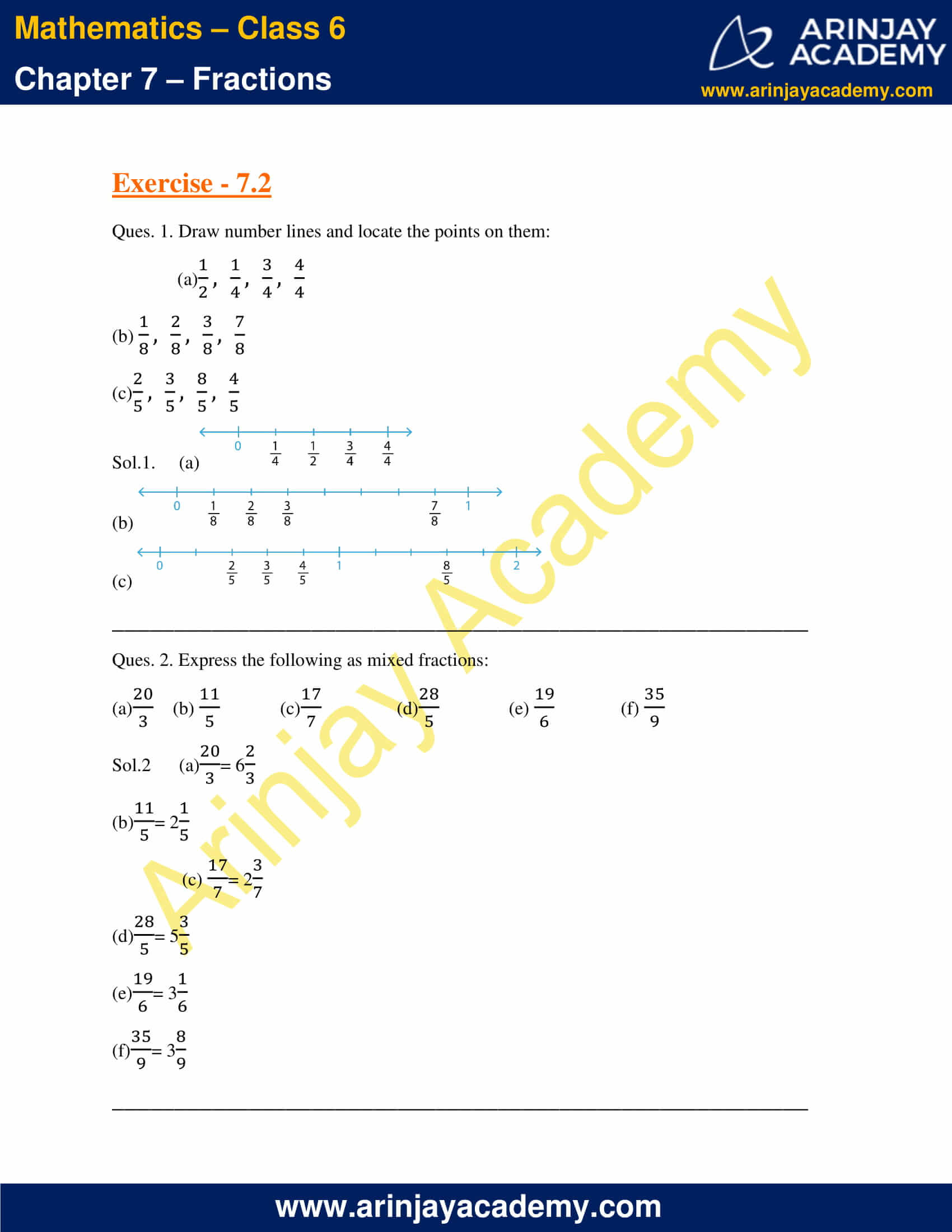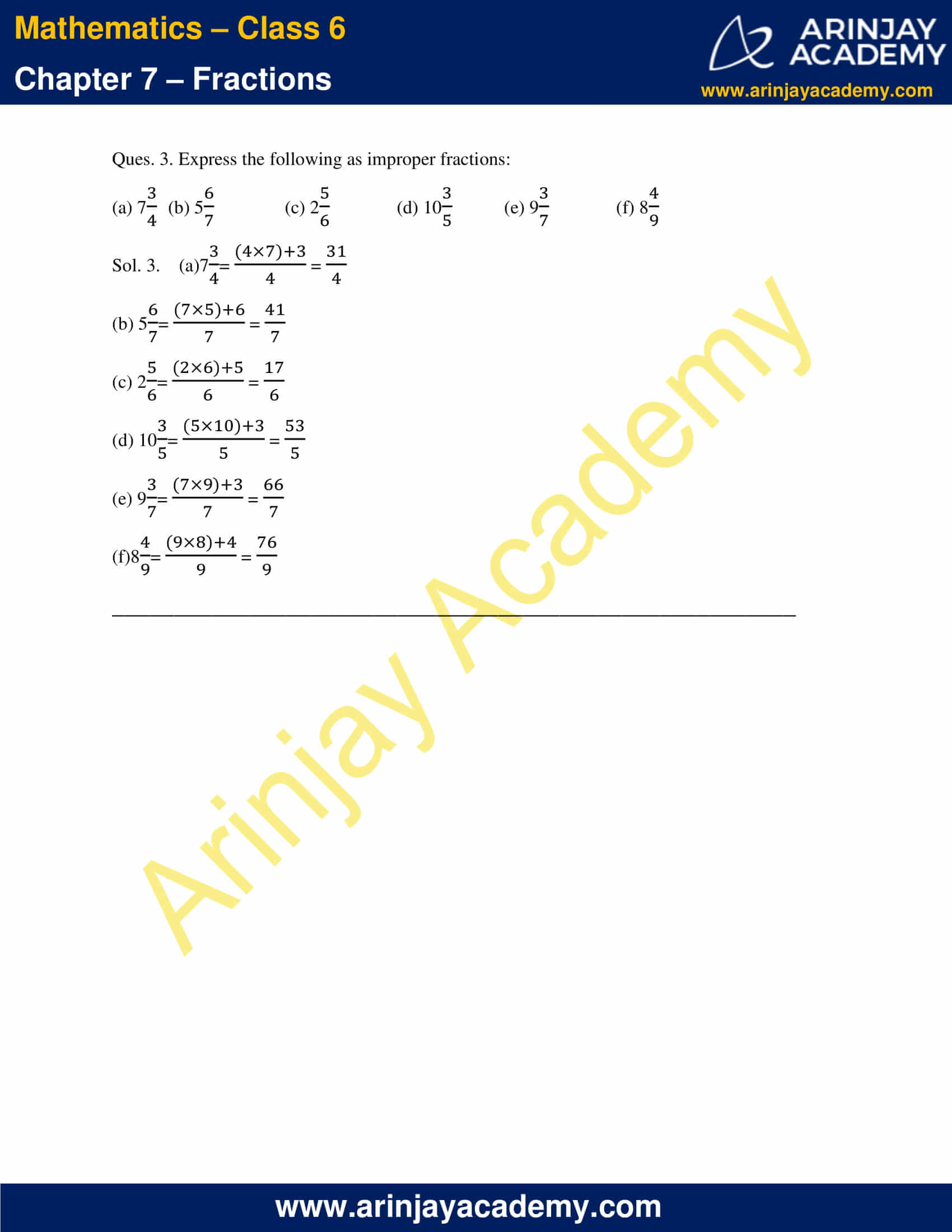### NCERT Solutions for Class 6 Maths Chapter 7 Exercise 7.3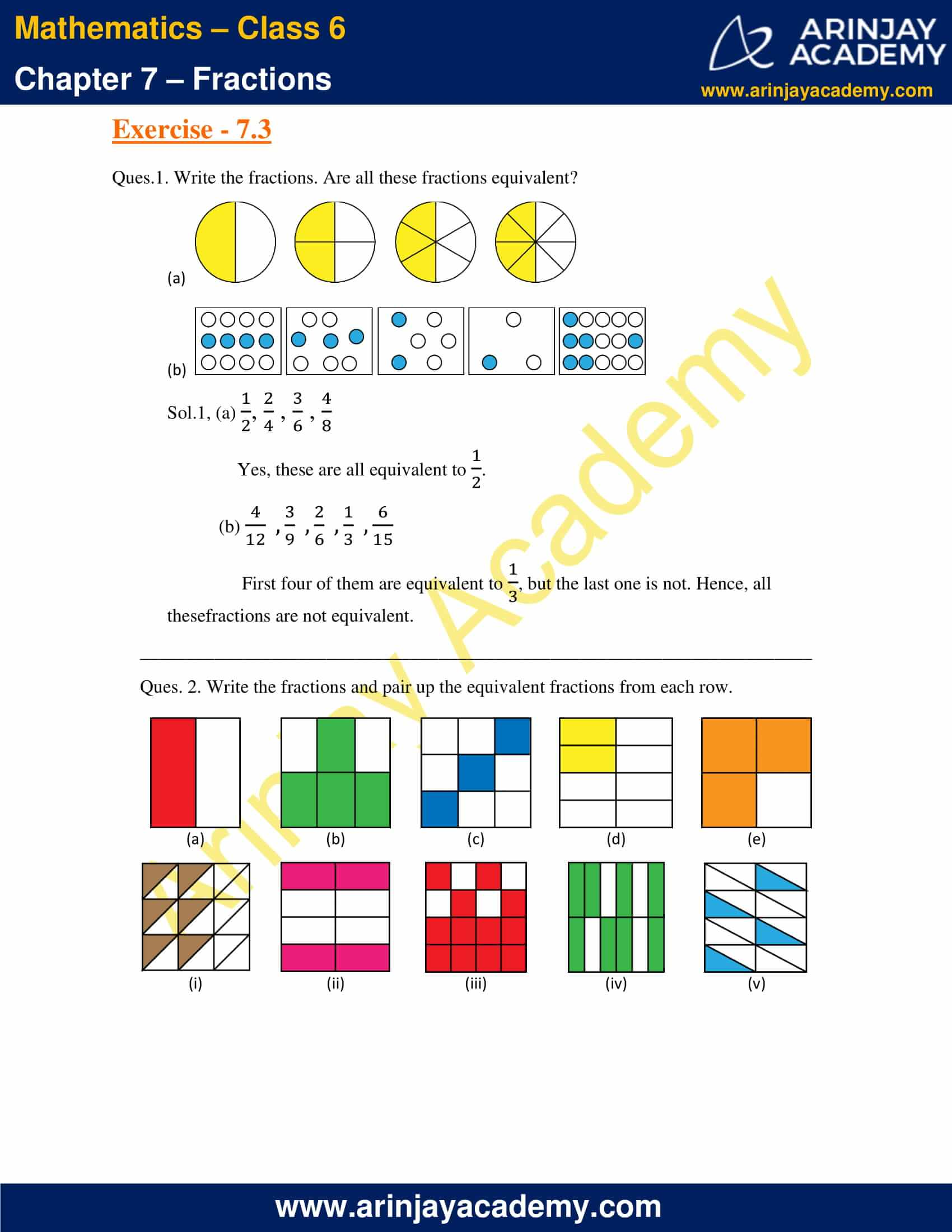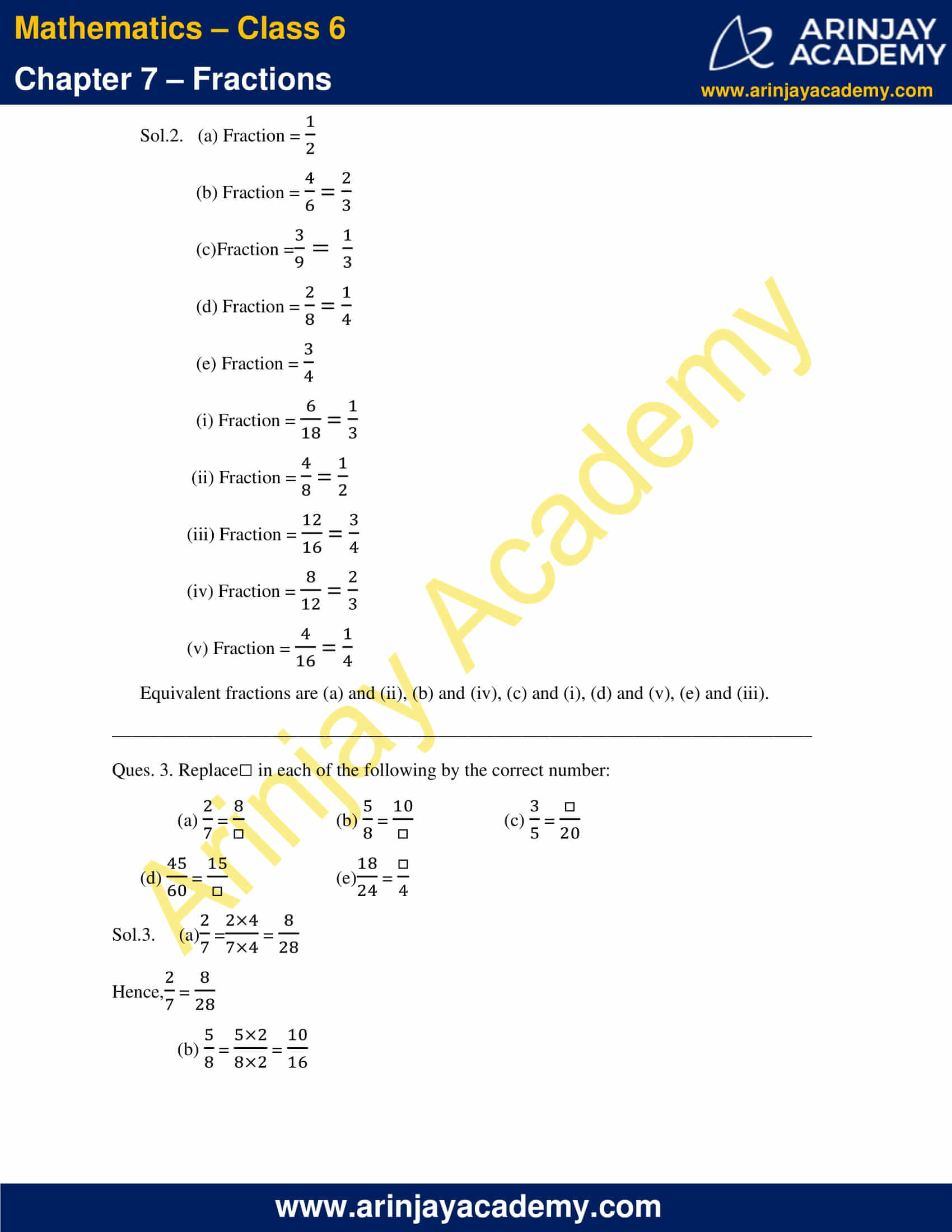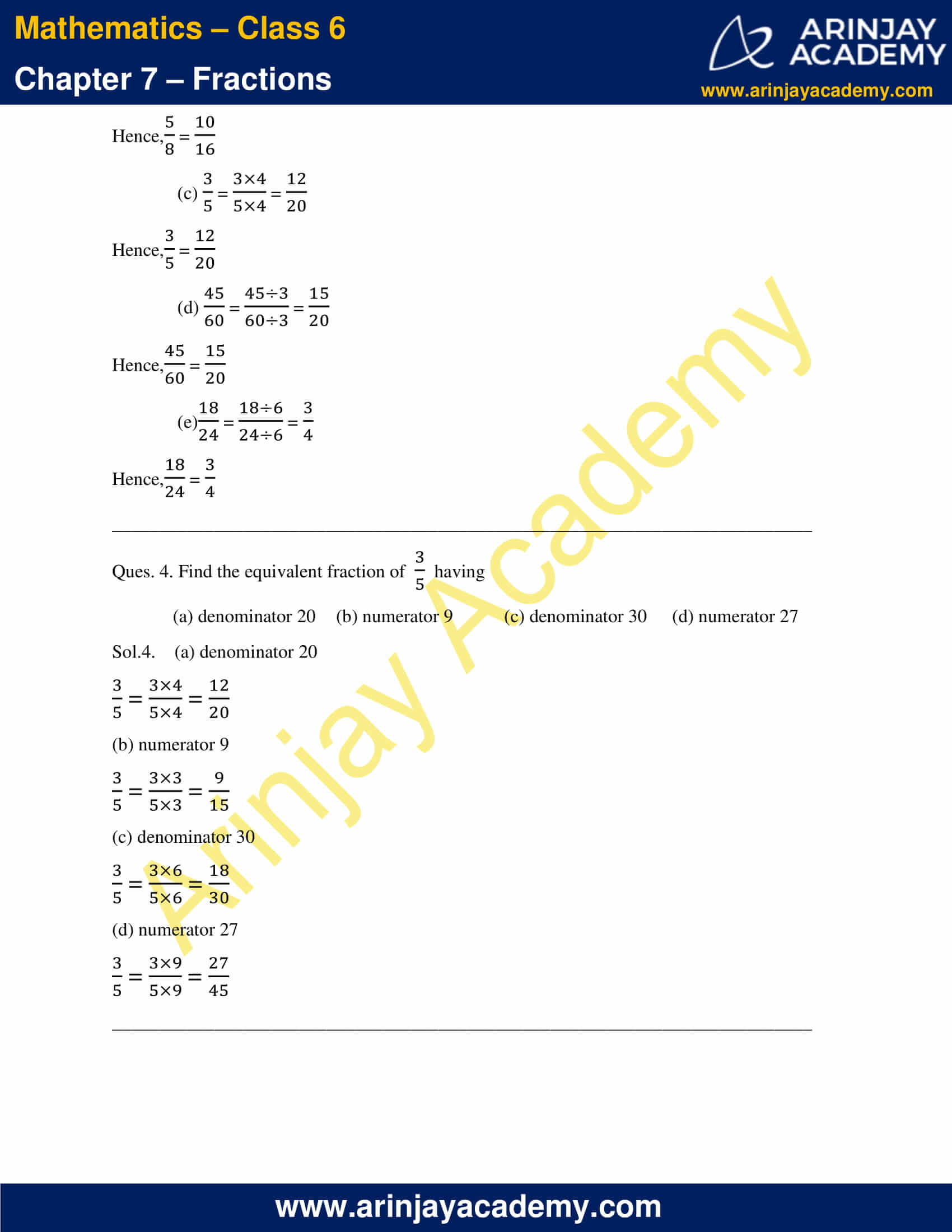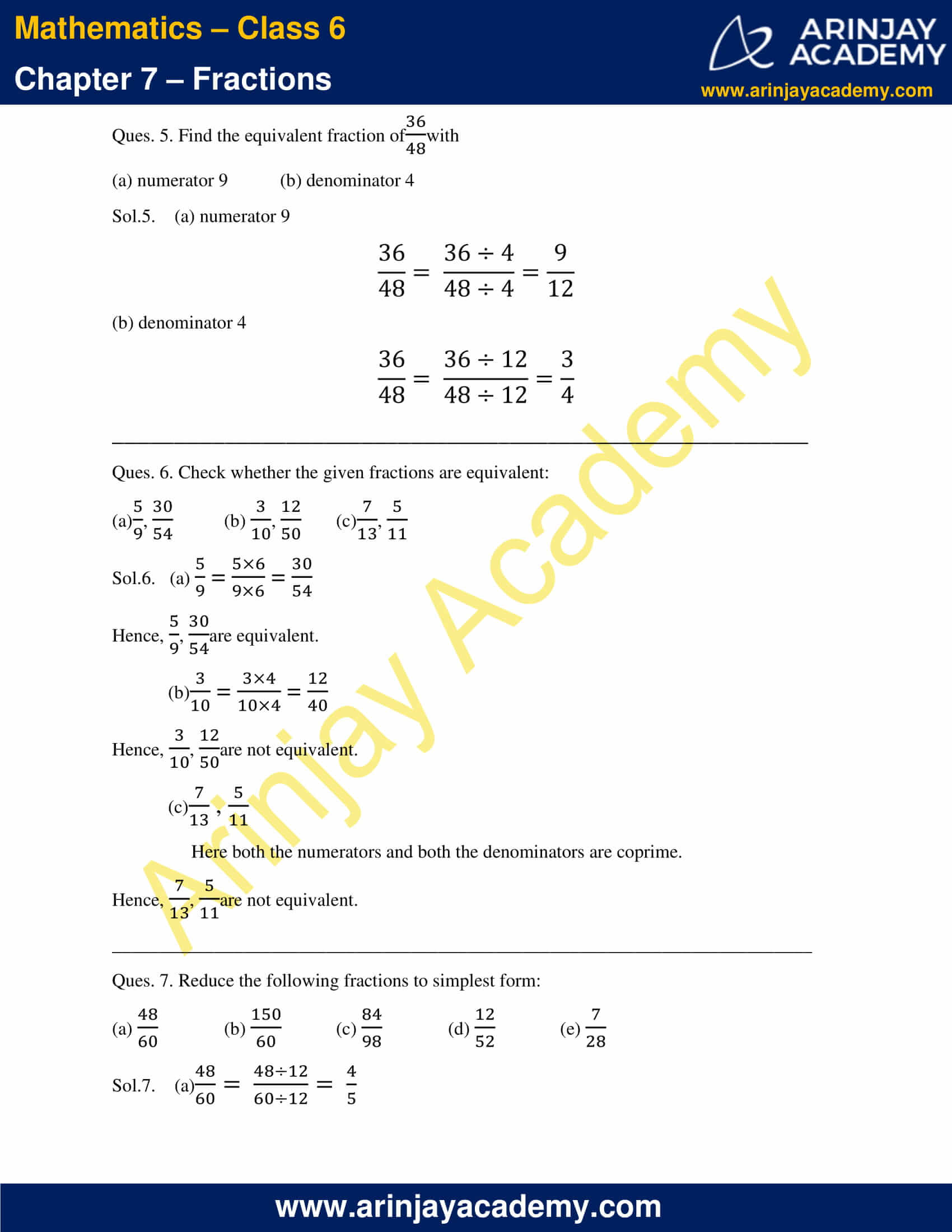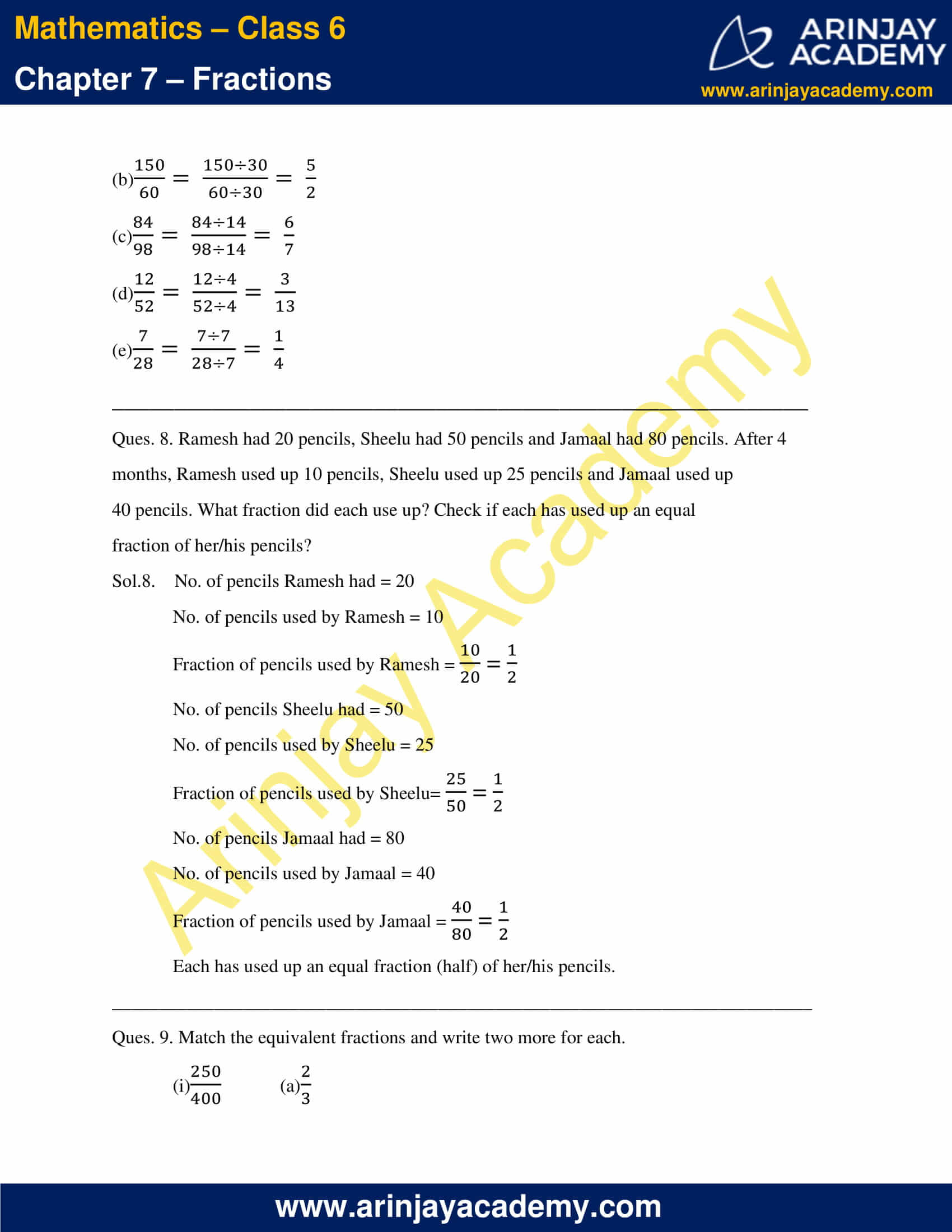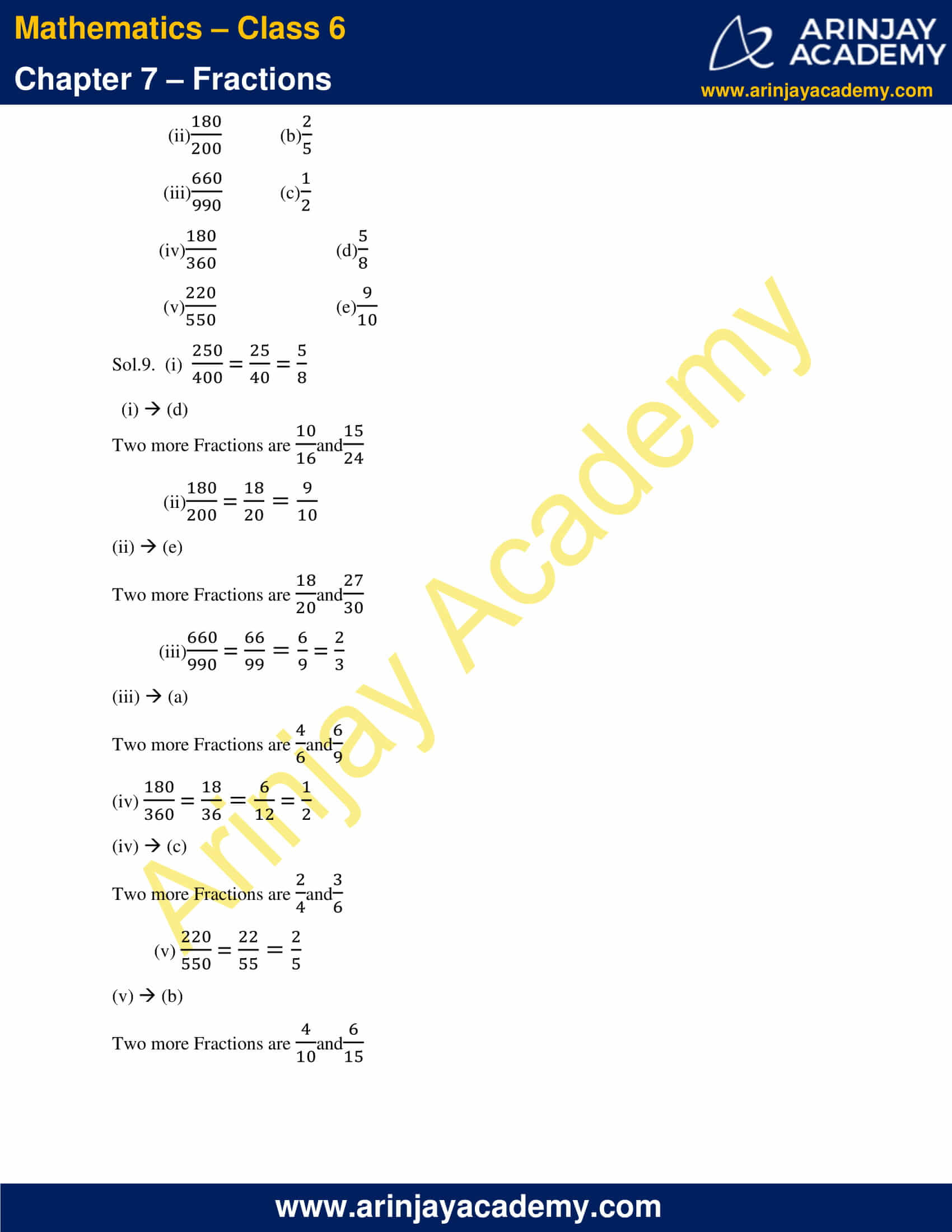### NCERT Solutions for Class 6 Maths Chapter 7 Exercise 7.4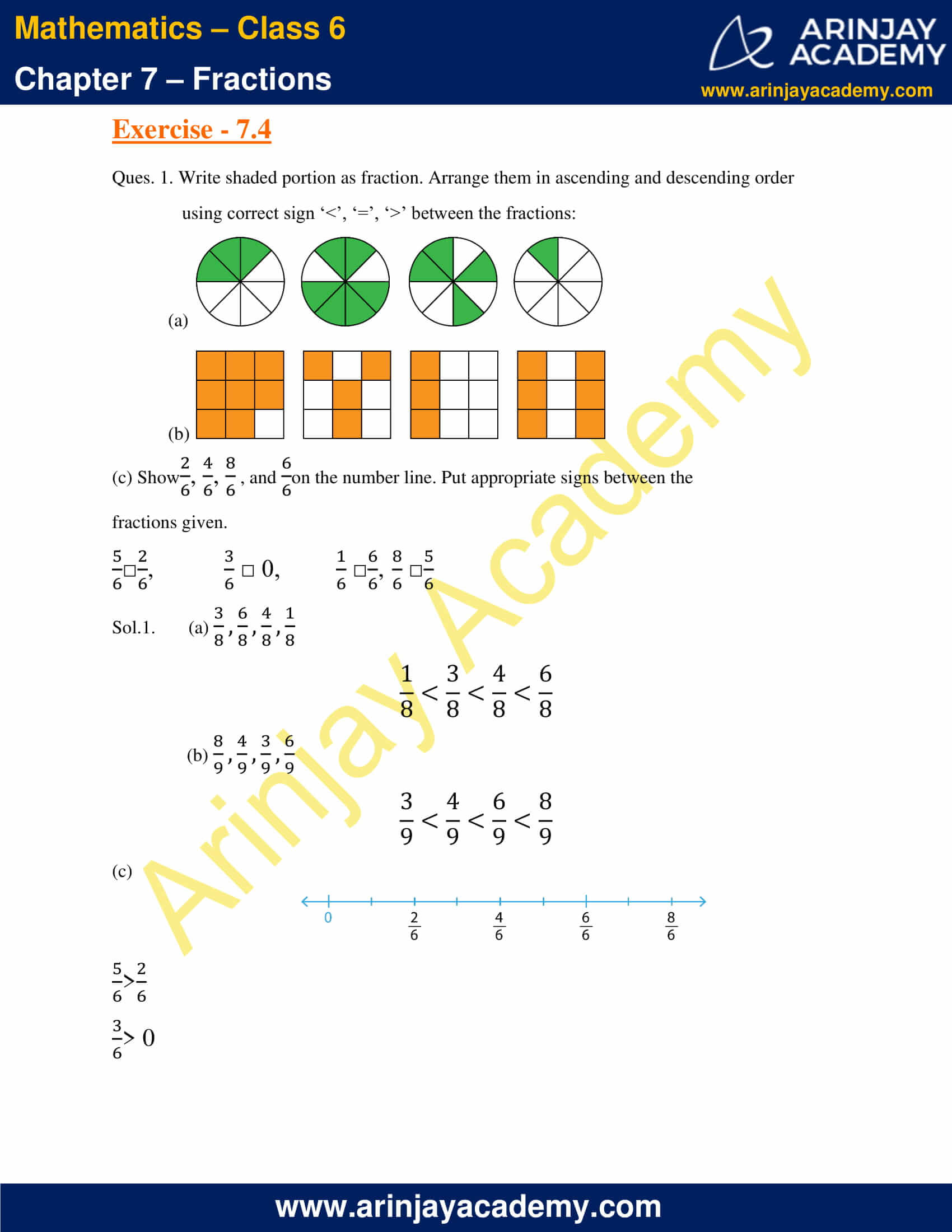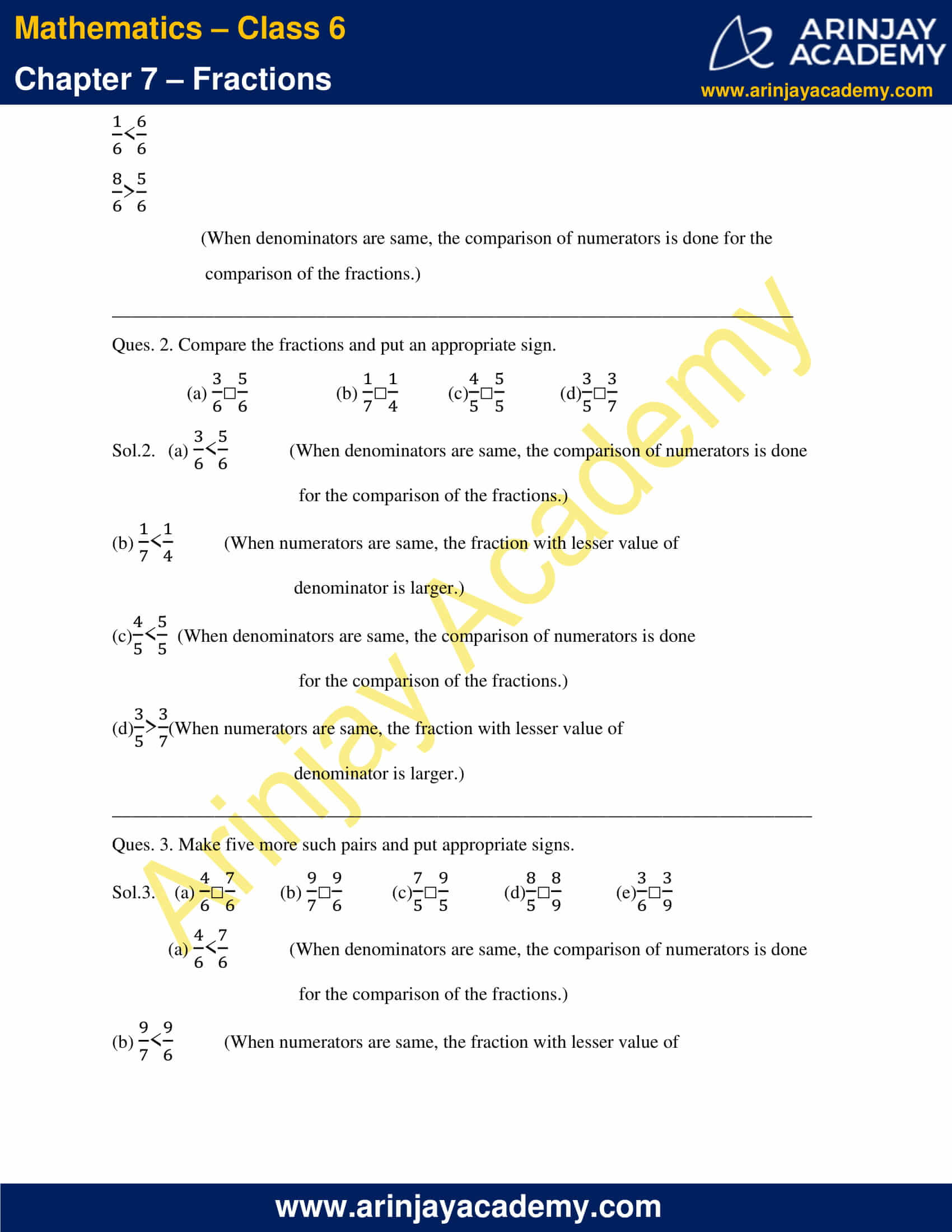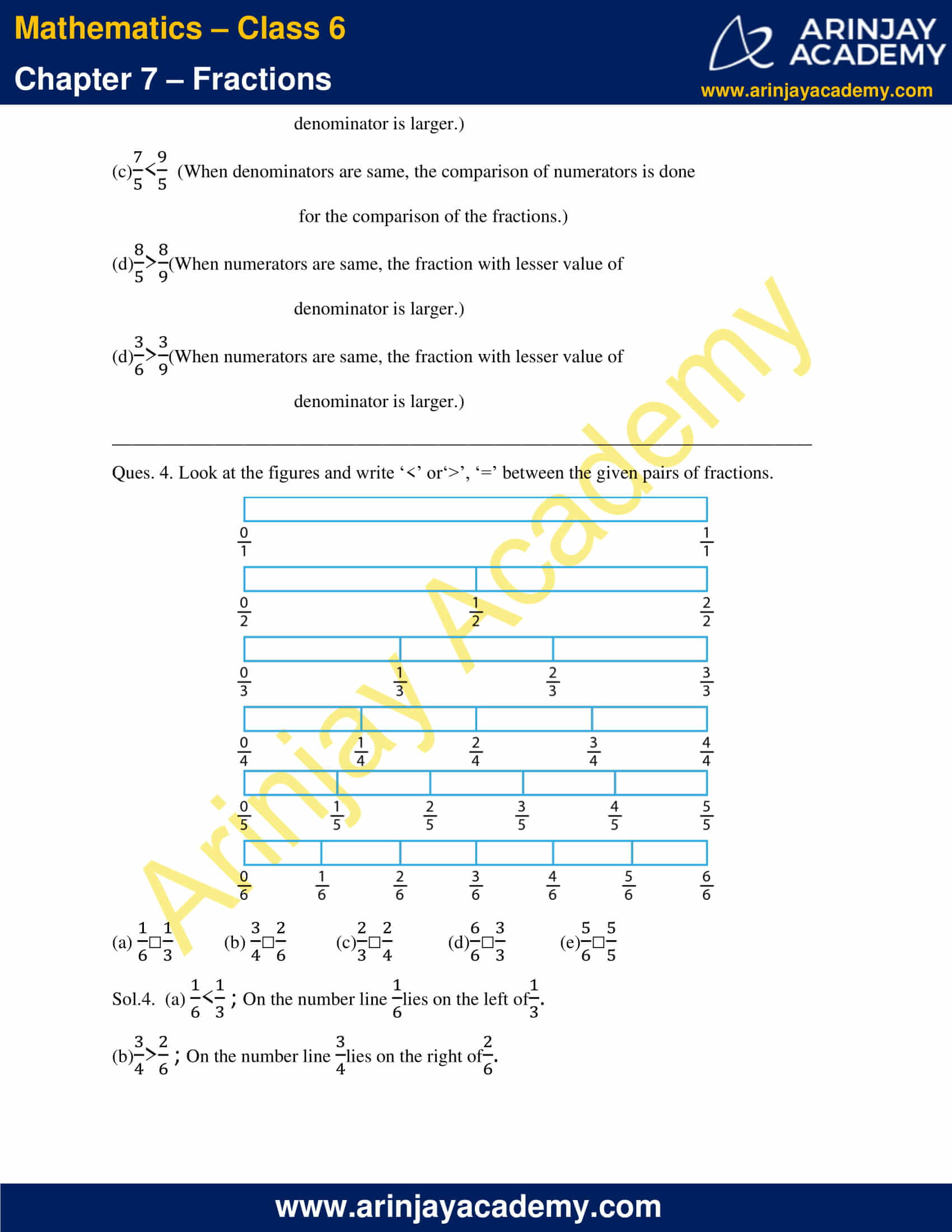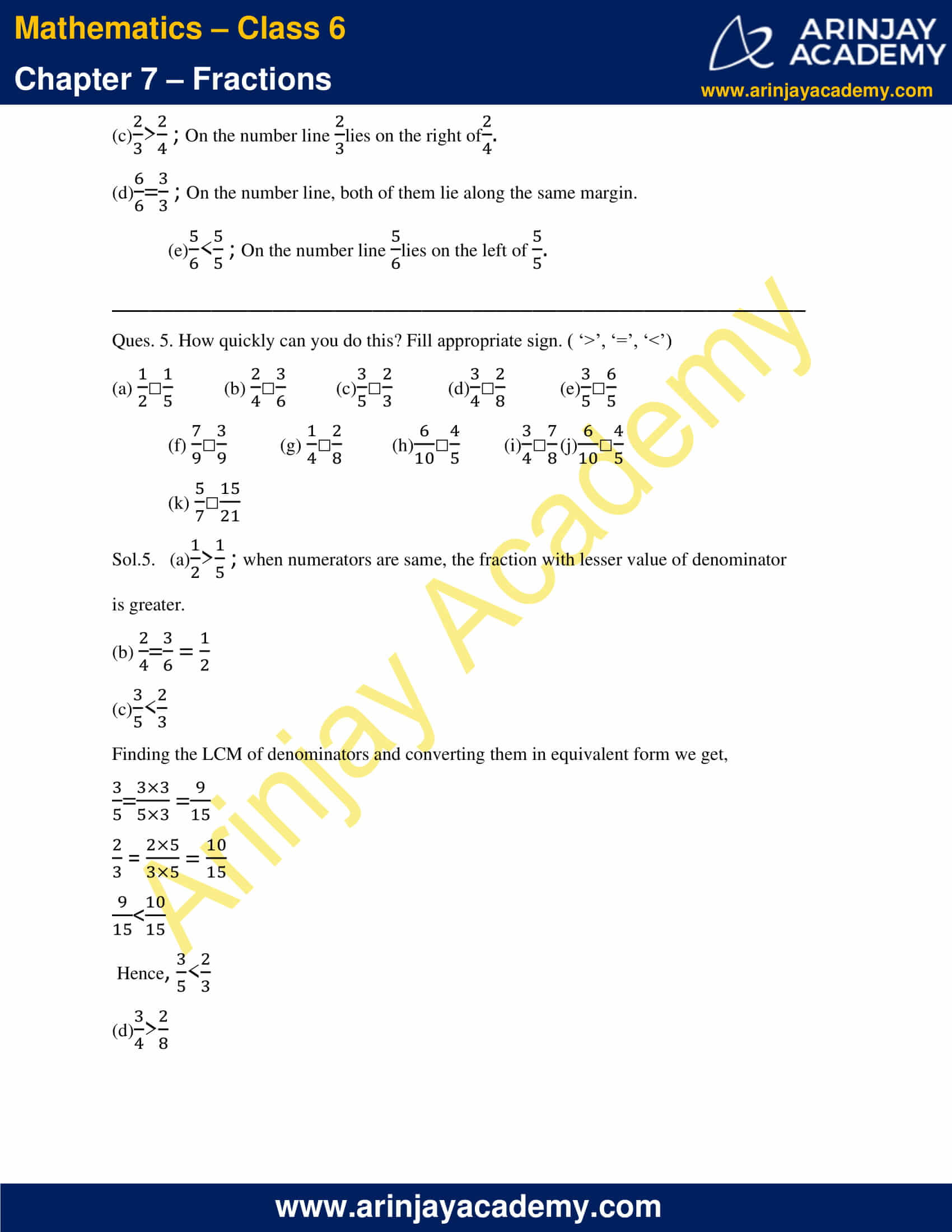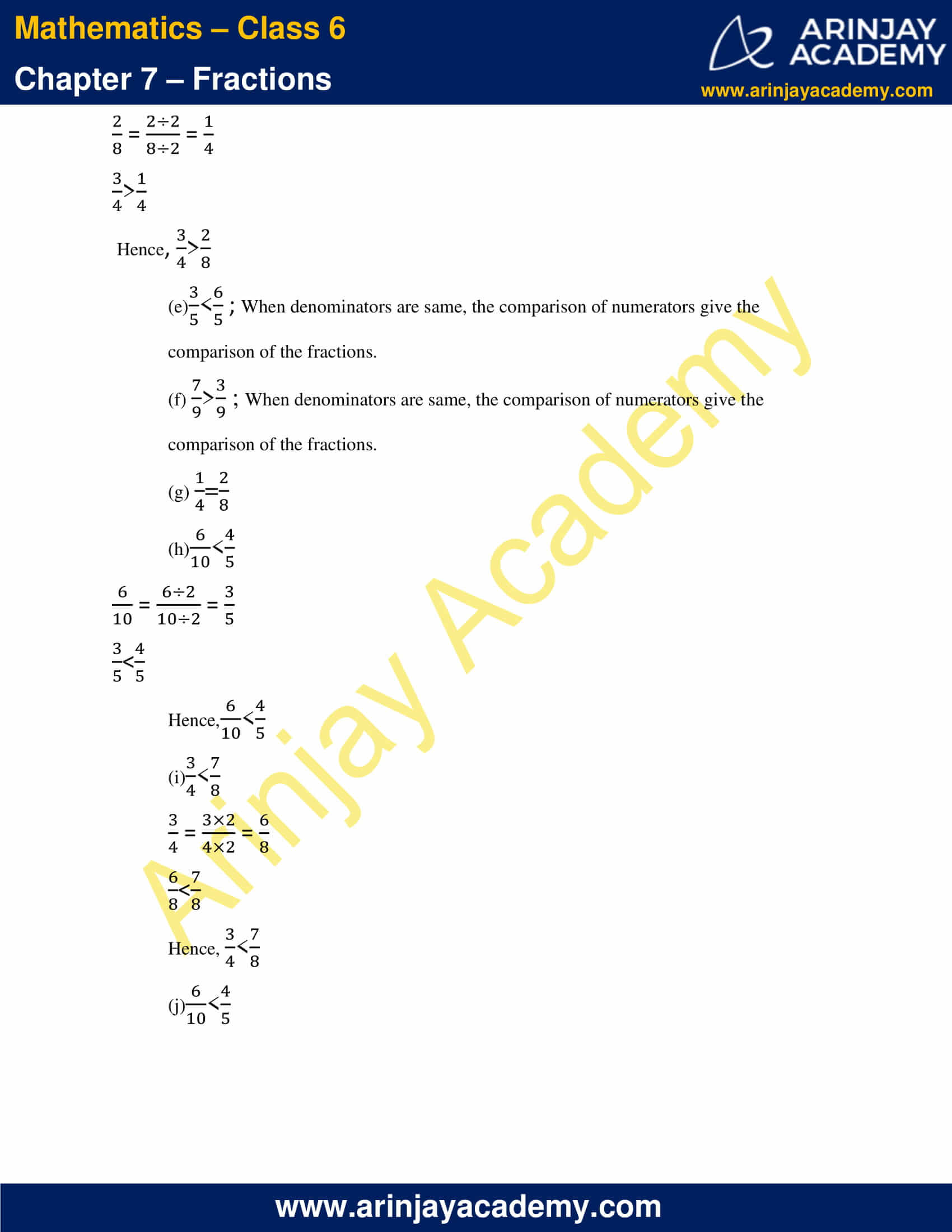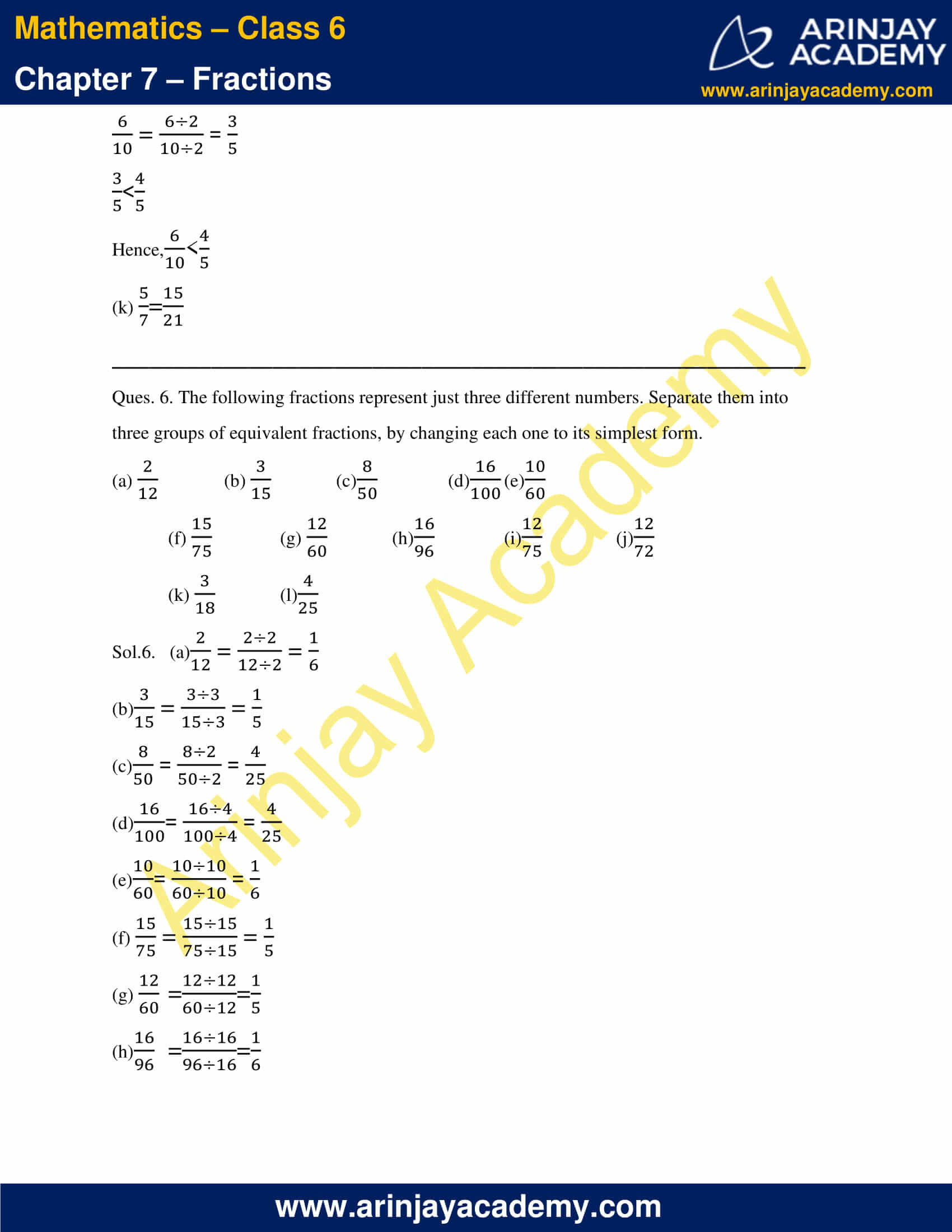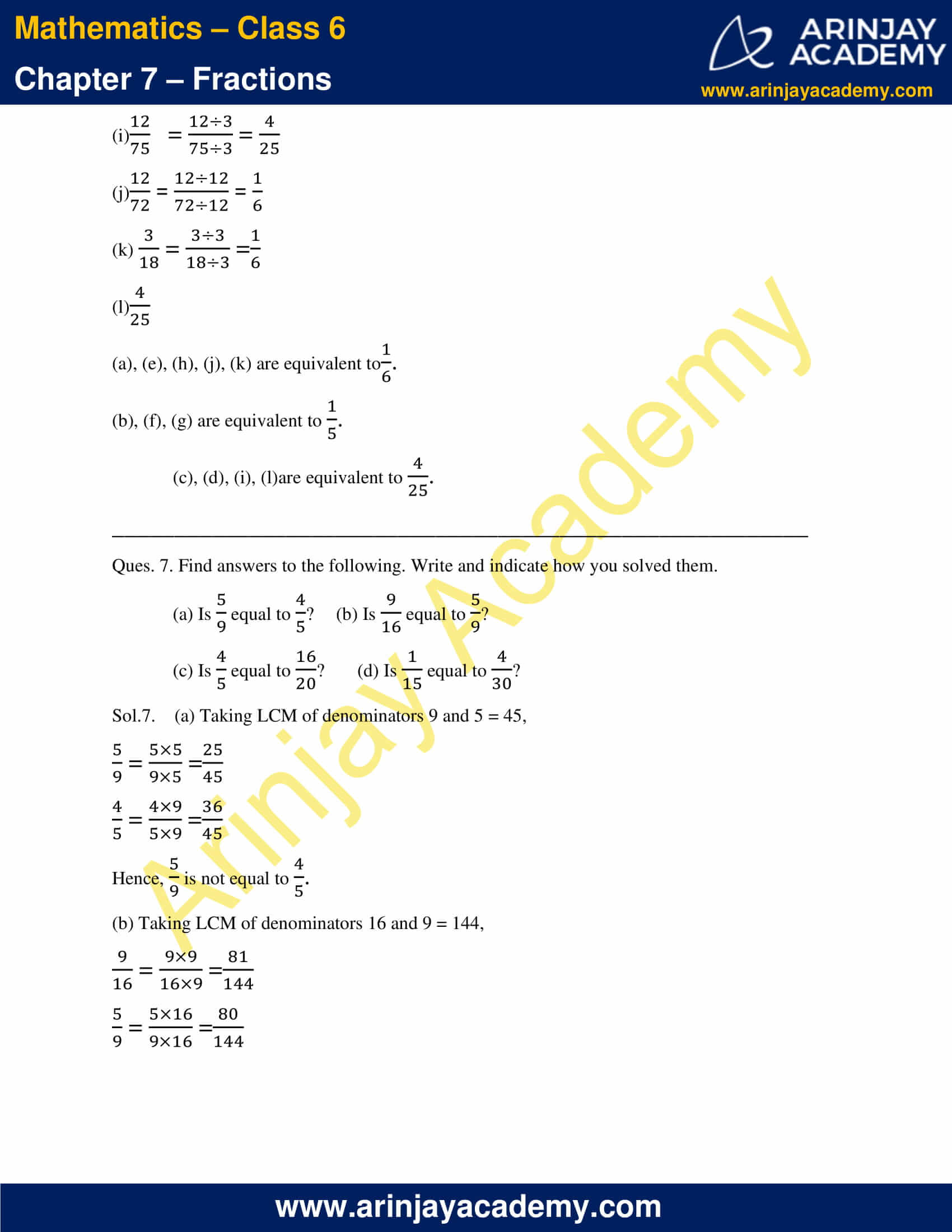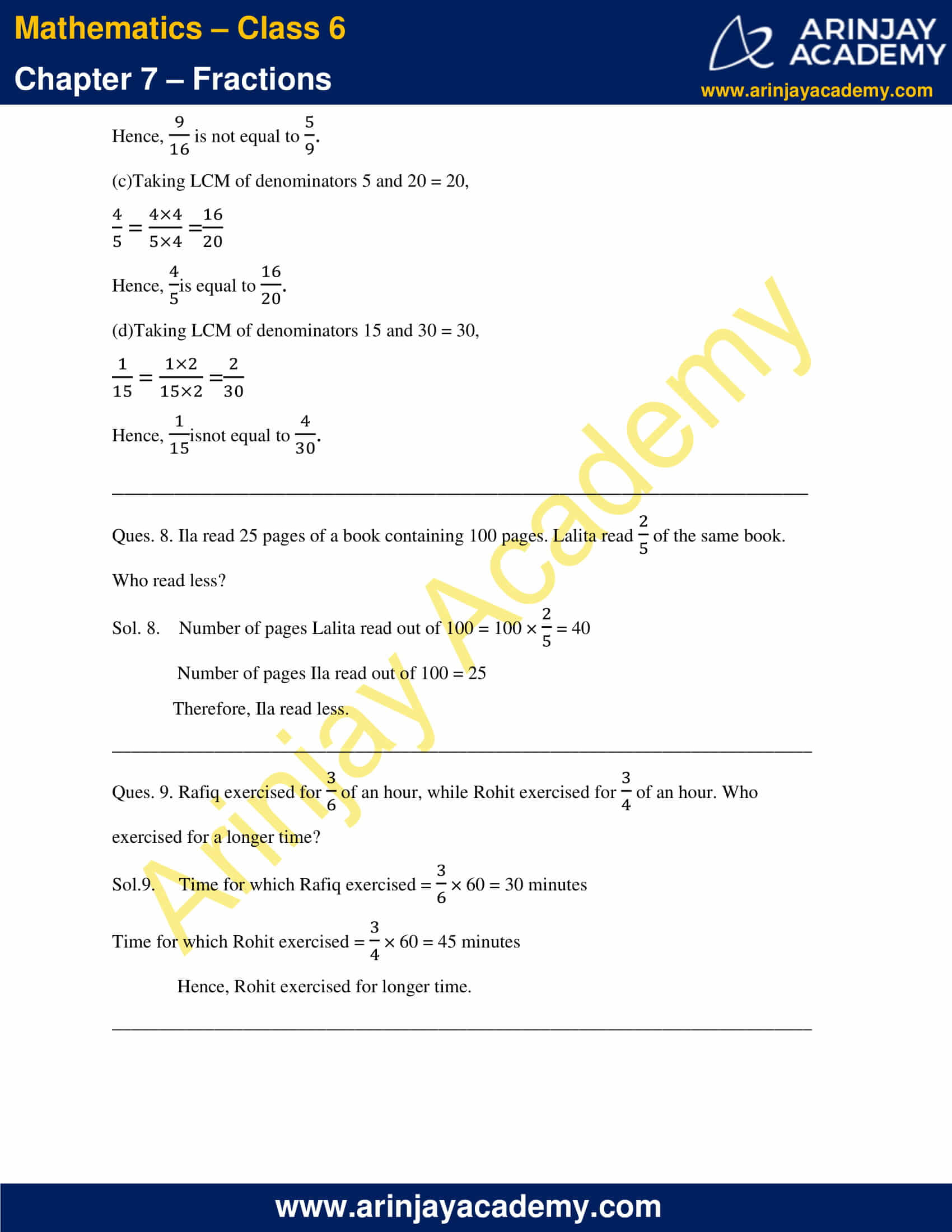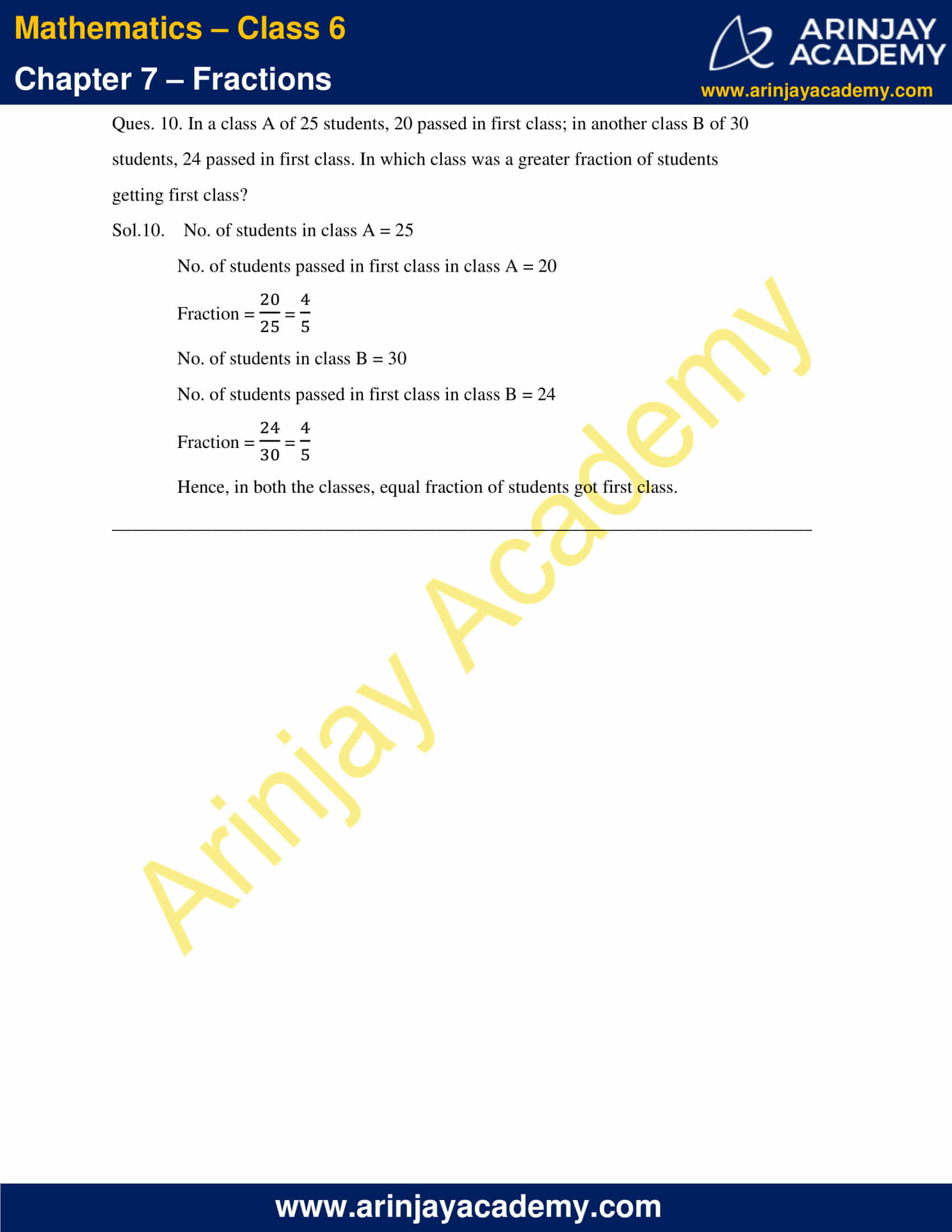### NCERT Solutions for Class 6 Maths Chapter 7 Exercise 7.5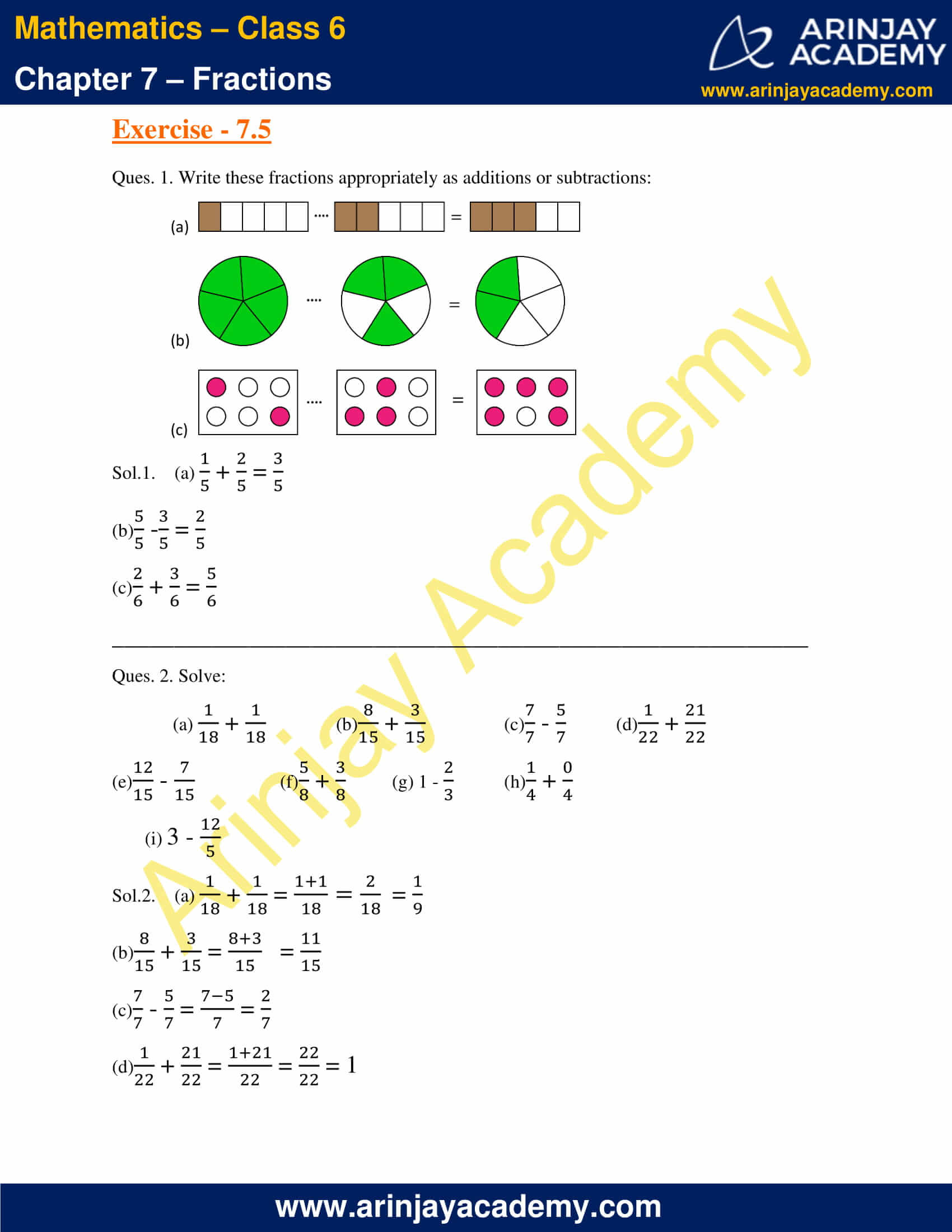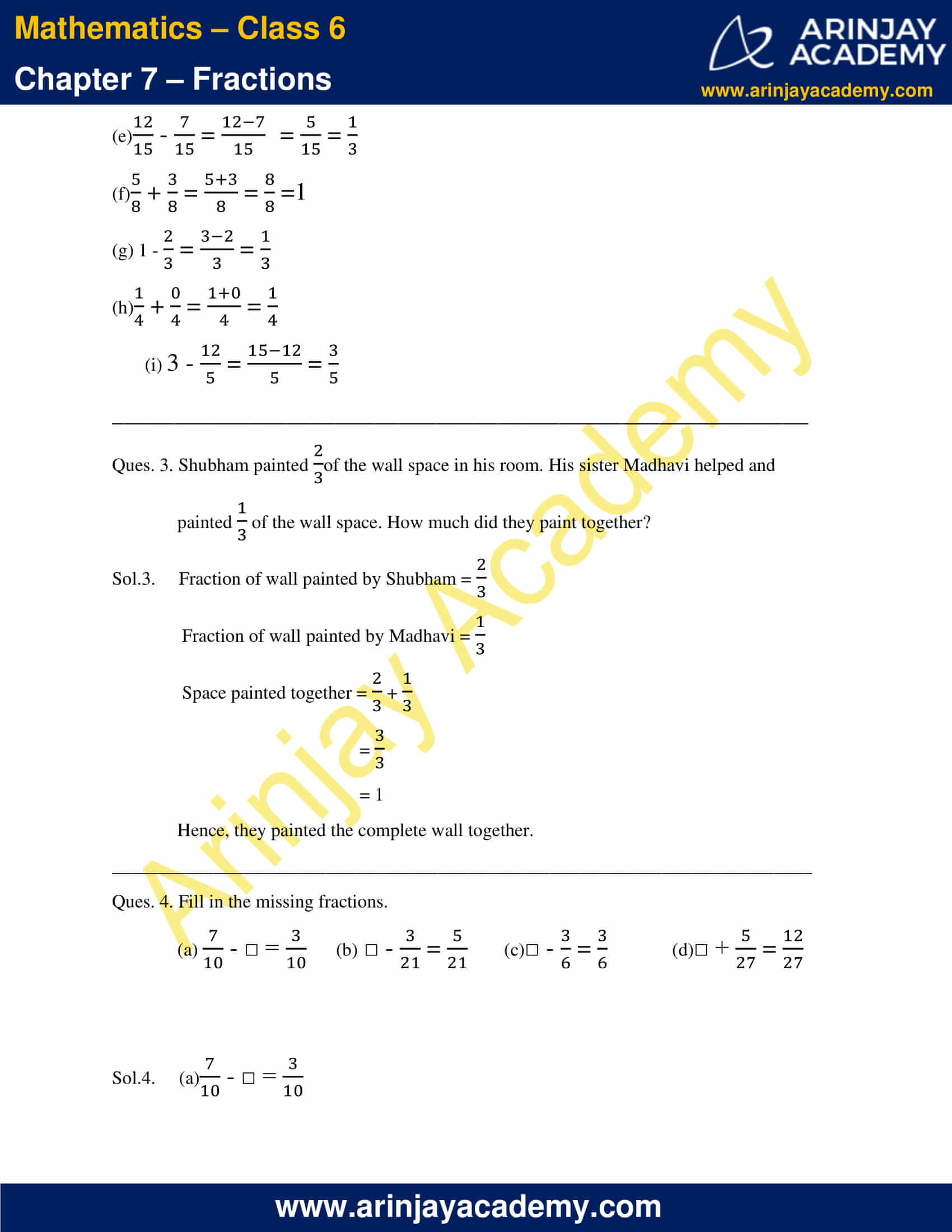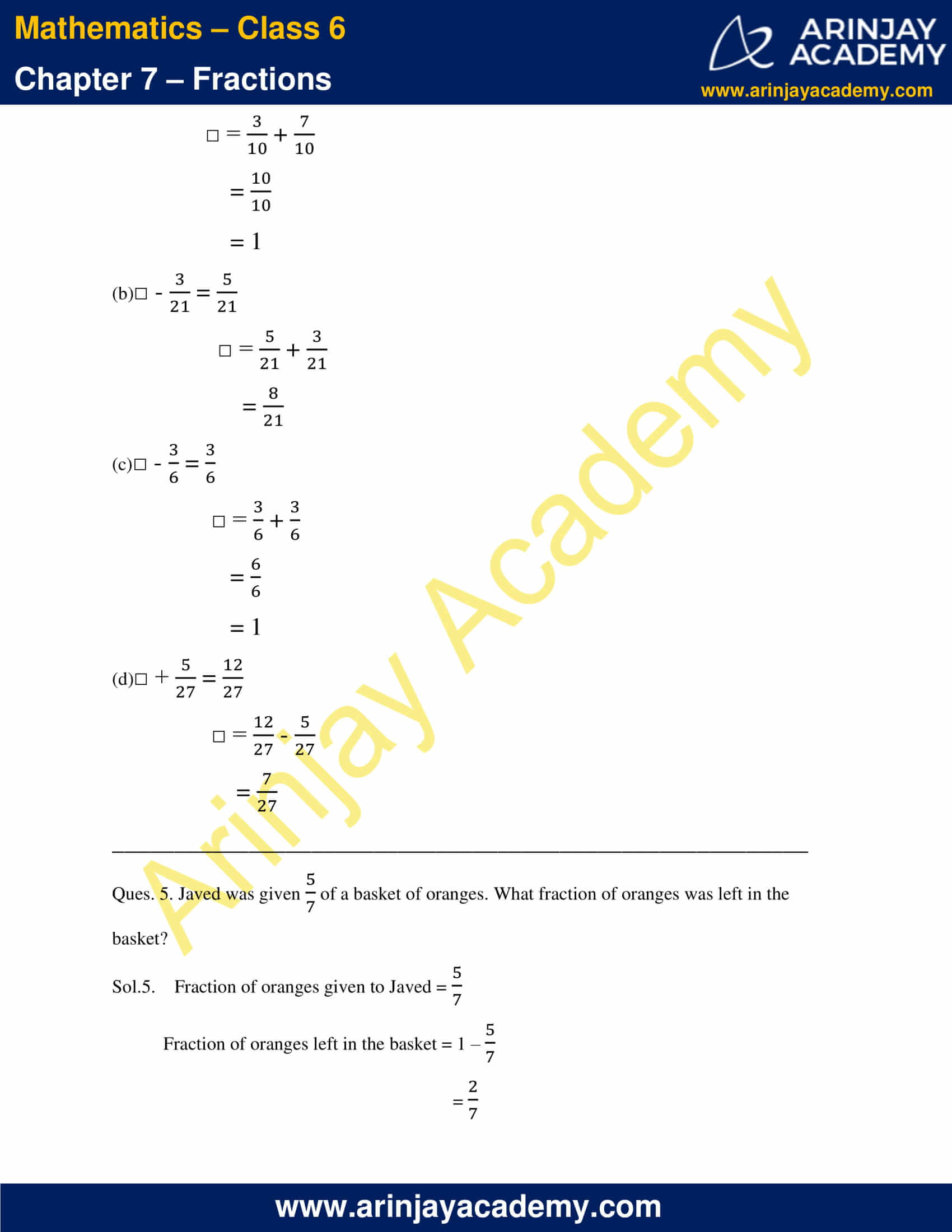### NCERT Solutions for Class 6 Maths Chapter 7 Exercise 7.6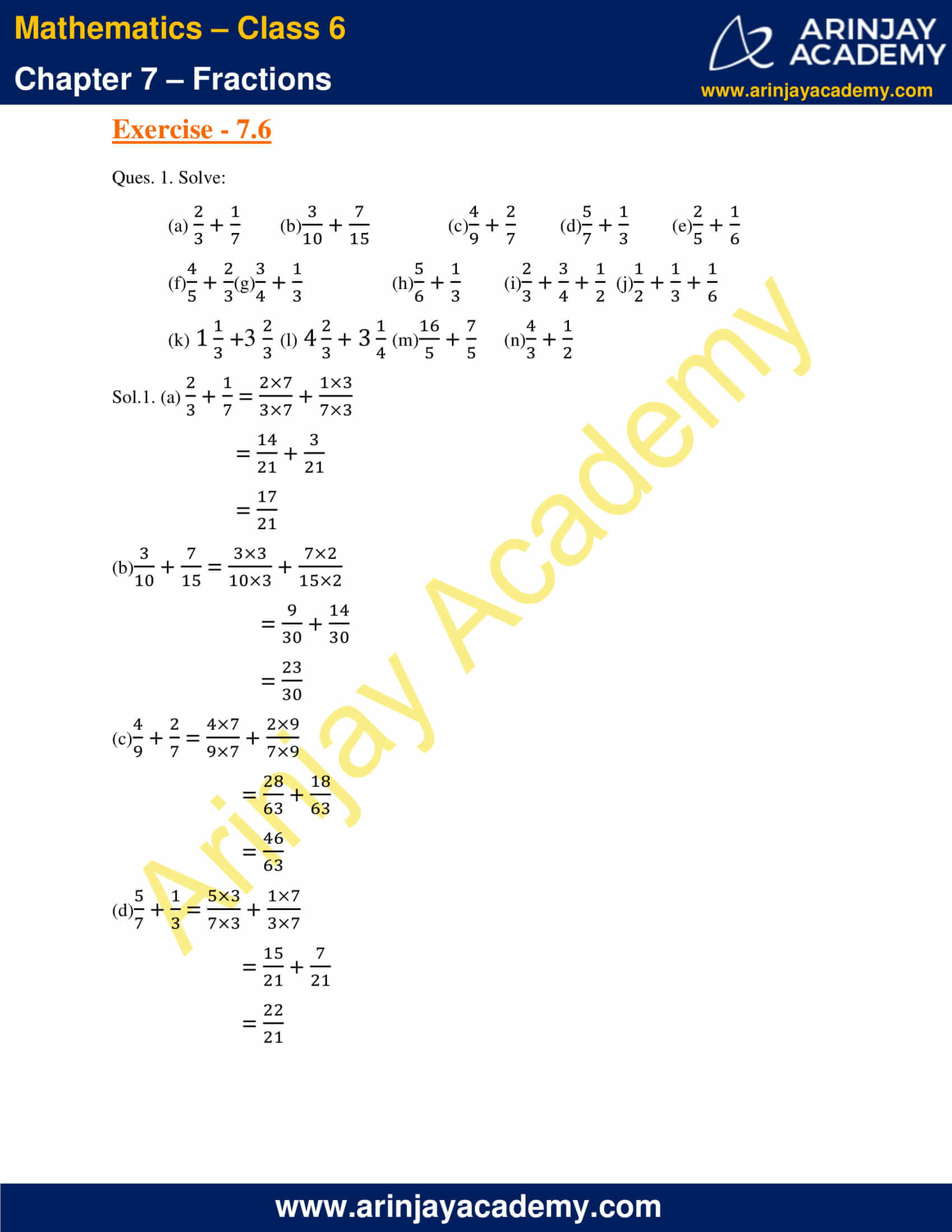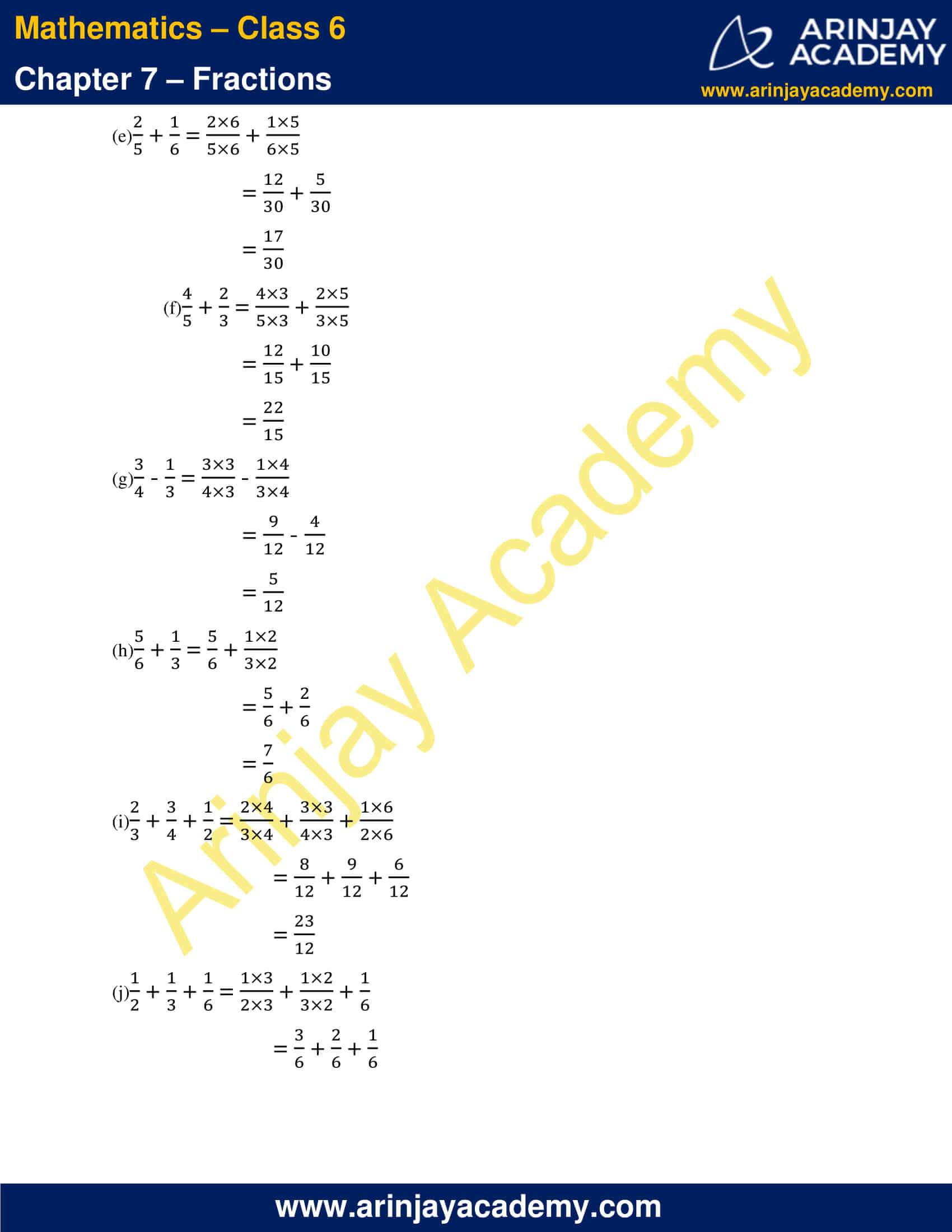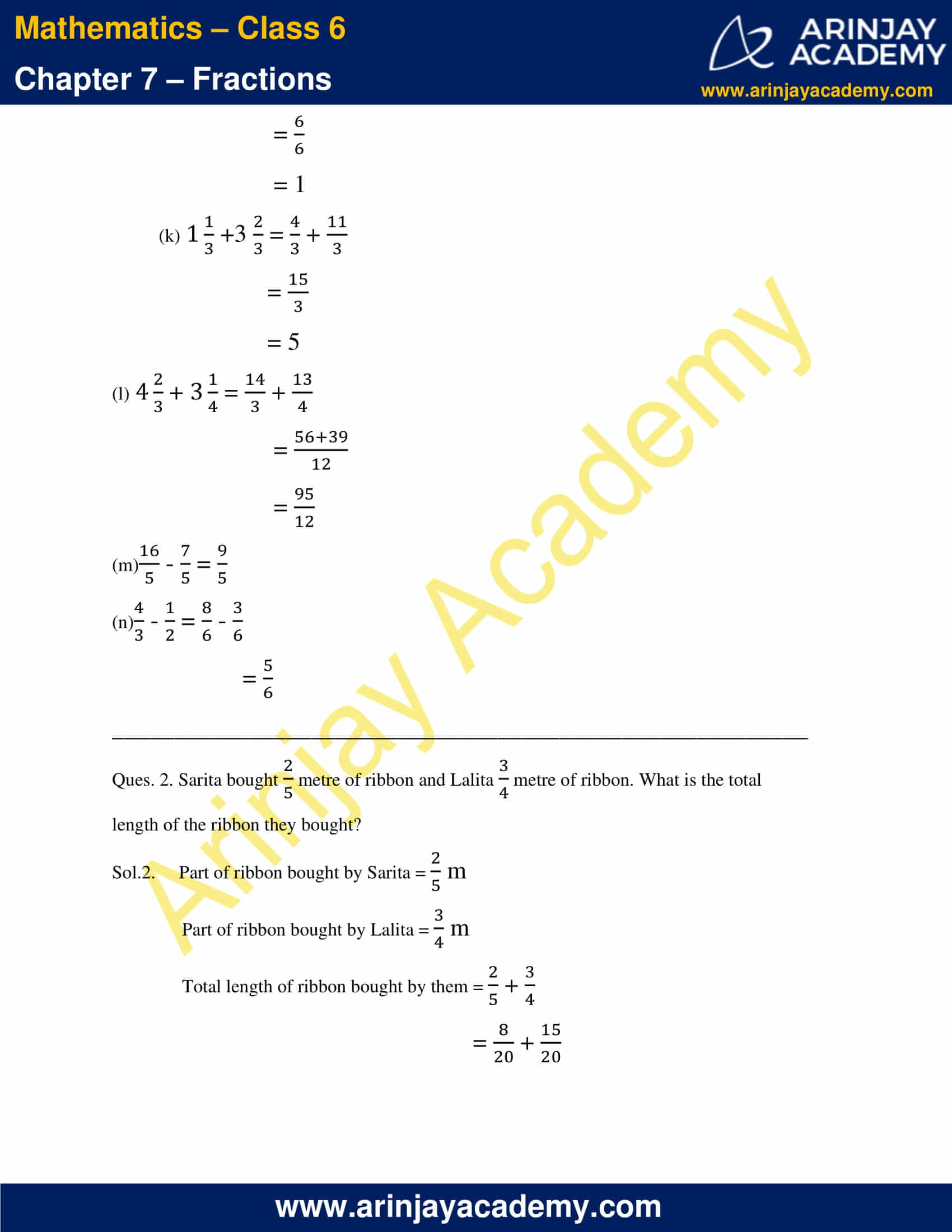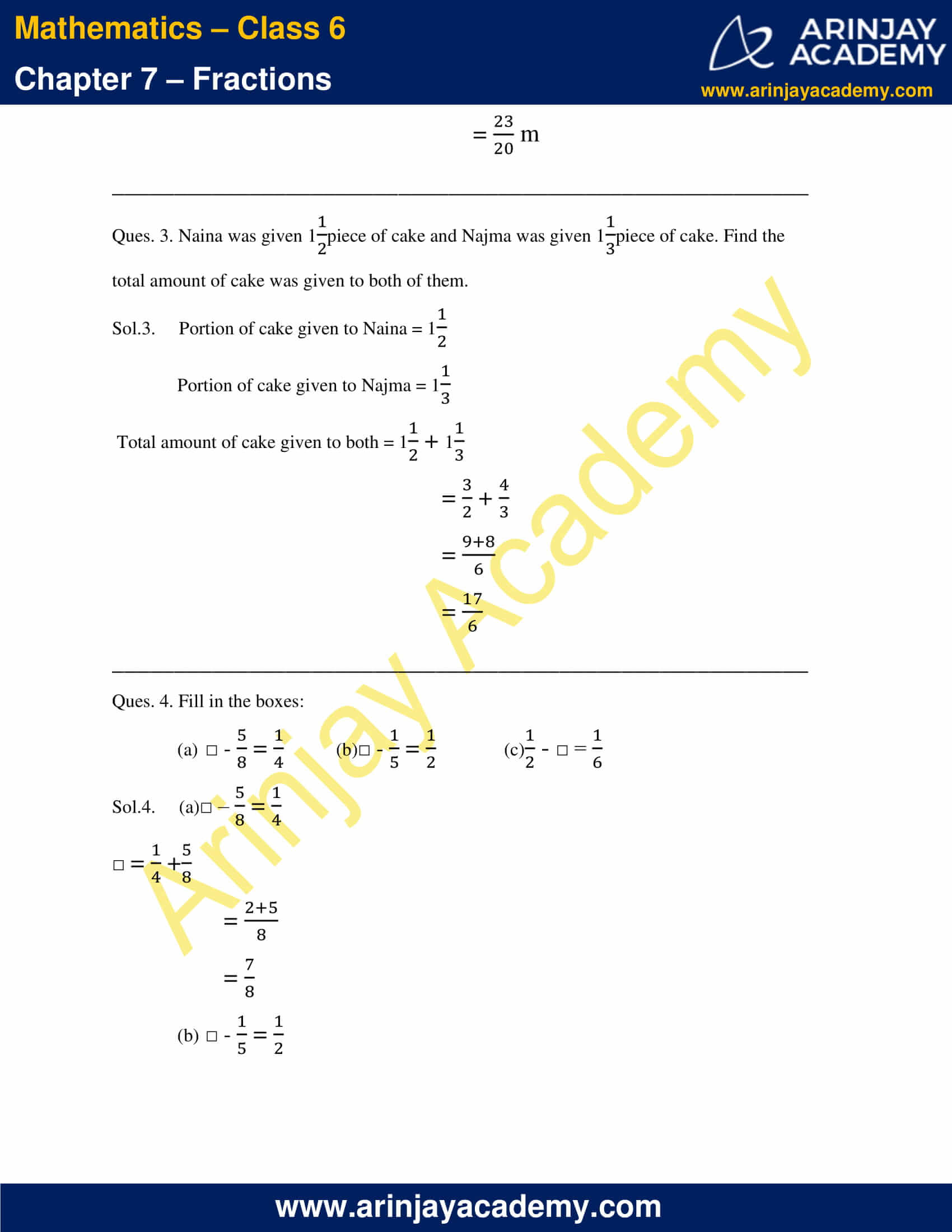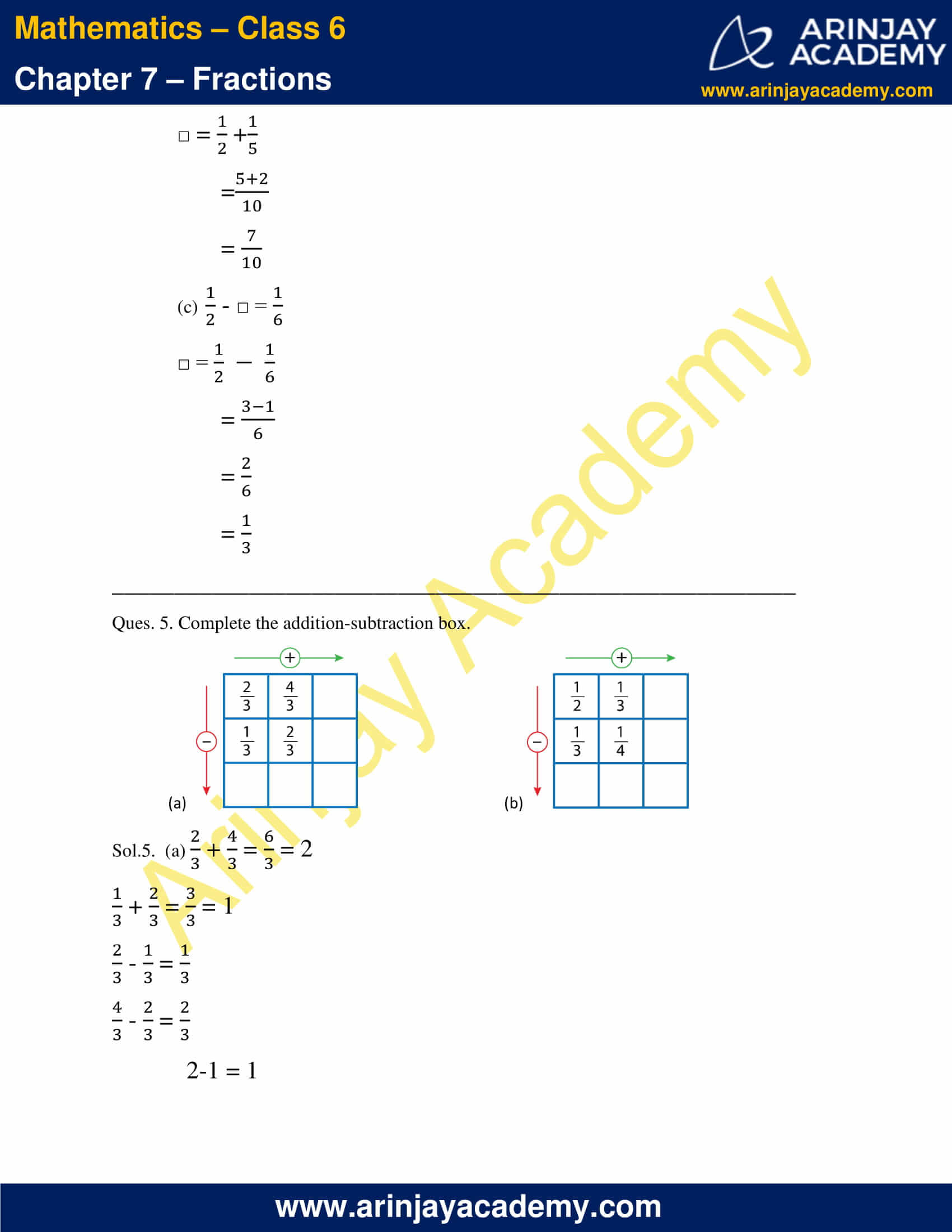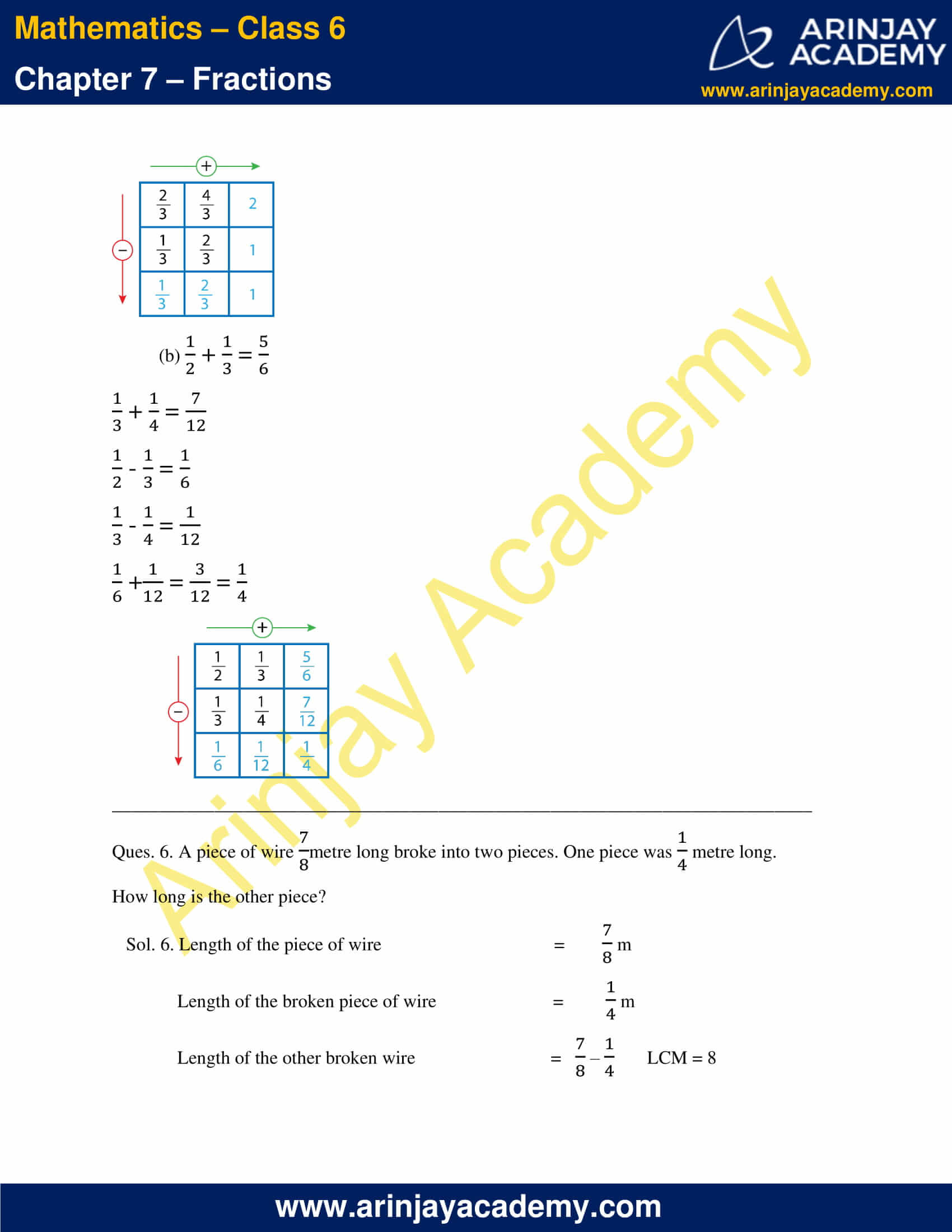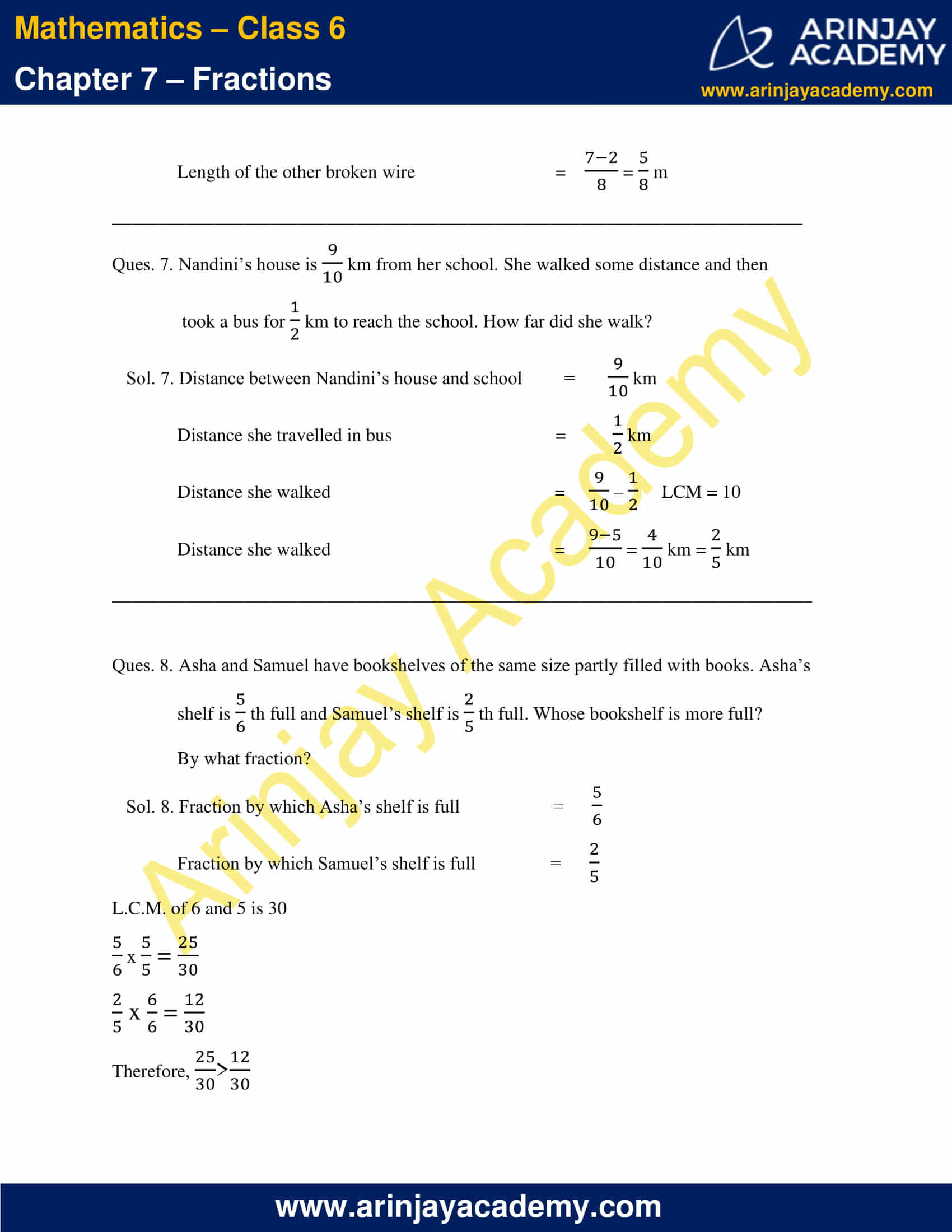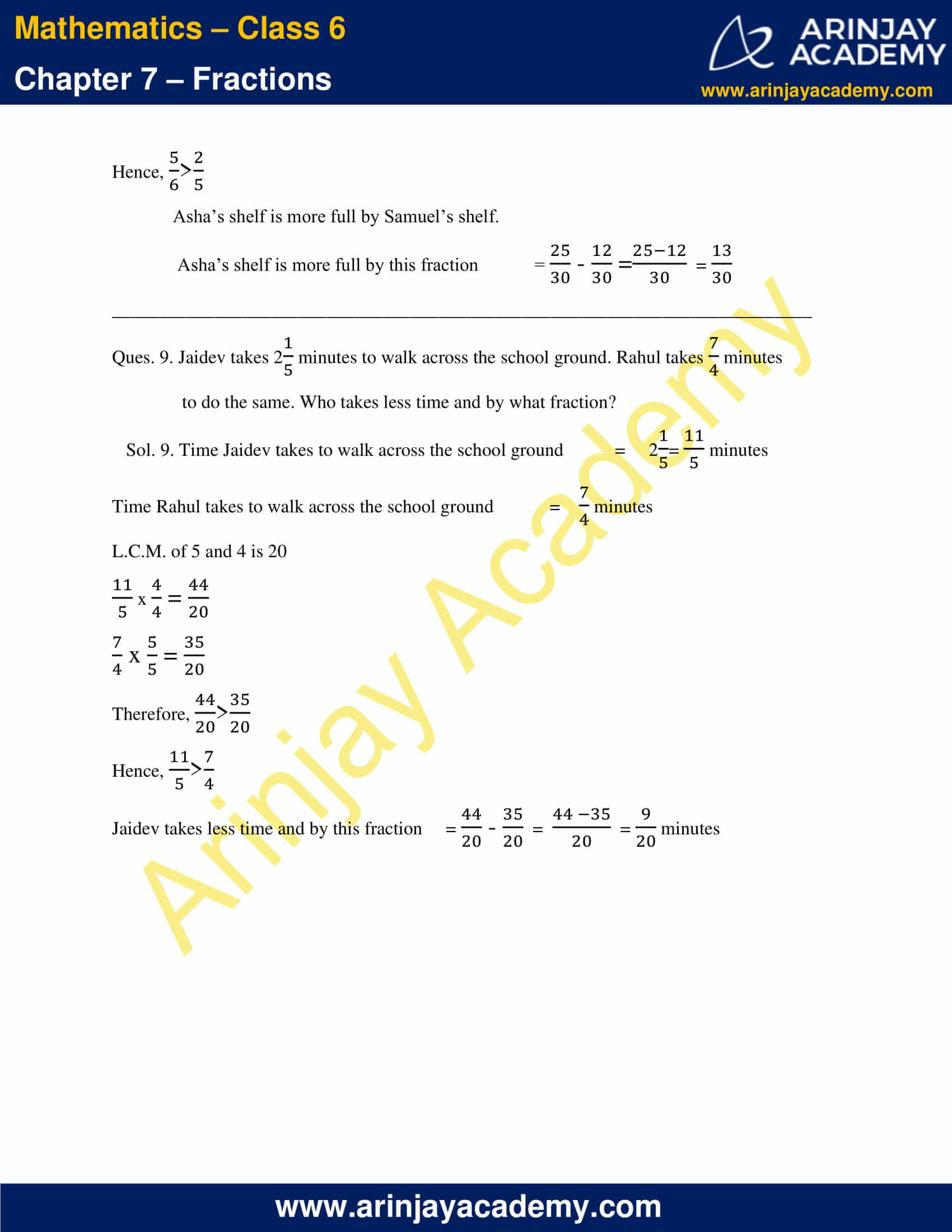### NCERT Solutions for Class 6 Maths Chapter 7 Exercise 7.1

Ques. 1. Write the fraction representing the shaded portion.

###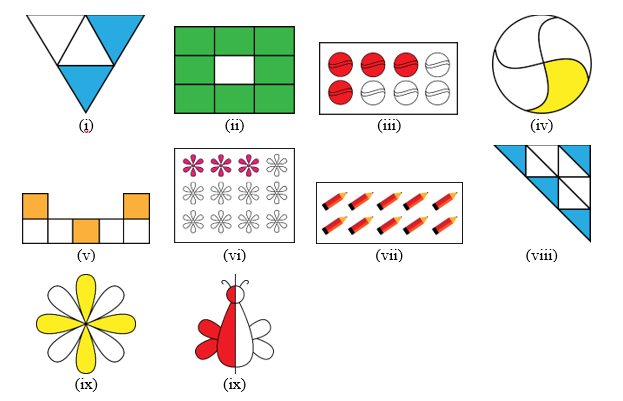Sol.1.  (i) Part of the triangle shaded = 2
Total parts of the triangle = 4
Fraction of shaded part =$\cfrac { 2 }{ 4 }$ =$\cfrac { 1 }{ 2 }$

(ii) Part of the rectangle shaded = 8
Total parts of the rectangle = 9
Fraction of shaded part =$\cfrac { 8 }{ 9 }$

(iii) Part of the figure shaded = 4
Total parts of the figure = 8
Fraction of shaded part =$\cfrac { 4 }{ 8 }$ =$\cfrac { 1 }{ 2 }$

(iv) Part of the circle shaded = 1
Total parts of the circle= 4
Fraction of shaded part =$\cfrac { 1 }{ 4 }$

(v) Part of the figure shaded = 3
Total parts of the figure = 7
Fraction of shaded part =$\cfrac { 3 }{ 7 }$

(vi) Part of the figure shaded = 3
Total parts of the figure = 12
Fraction of shaded part =$\cfrac { 3 }{ 12 }$ =$\cfrac { 1 }{ 4 }$

(vii) Part of the figure shaded = 10
Total parts of the figure = 10
Fraction of shaded part =$\cfrac { 10 }{ 10 }$ = 1

(viii) Part of the figure shaded = 4
Total parts of the figure = 9
Fraction of shaded part =$\cfrac { 4 }{ 9 }$

(ix) Part of the figure shaded = 4
Total parts of the figure = 8
Fraction of shaded part =$\cfrac { 4 }{ 8 }$ =$\cfrac { 1 }{ 2 }$

(x) Part of the figure shaded = 4
Total parts of the figure = 8
Fraction of shaded part =$\cfrac { 4 }{ 8 }$ =$\cfrac { 1 }{ 2 }$

Ques. 2. Colour the part according to the given fraction.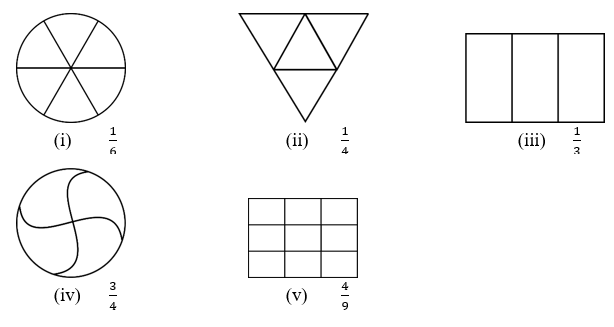Solution: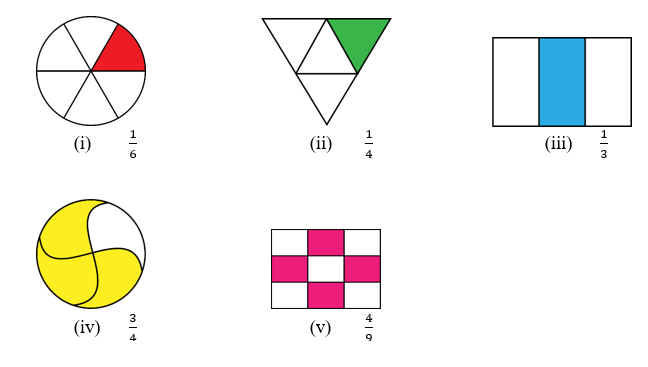Ques. 3. Identify the error, if any.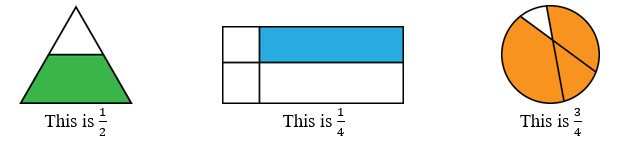Sol.3.   In all the three cases, the shaded portions do not represent the given fractions.
For correct shading of portions as per the given fractions, the figure should be divided into equal parts and then shaded.

Ques. 4. What fraction of a day is 8 hours?

Sol.4. No. of hours in a day = 24
No. of hours given = 8
Fraction =$\cfrac { 8 }{ 24 }$ =$\cfrac { 1 }{ 3 }$

Ques. 5. What fraction of an hour is 40 minutes?
Sol.5. No. of minutes in an hour = 60
No. of minutes given = 40
Fraction =$\cfrac { 40 }{ 60 }$ =$\cfrac { 2 }{ 3 }$

Ques. 6. Arya, Abhimanyu, and Vivek shared lunch. Arya has brought two sandwiches, one made of vegetable and one of jam. The other two boys forgot to bring their lunch. Arya agreed to share his sandwiches so that each person will have an equal share of each sandwich.
(a) How can Arya divide his sandwiches so that each person has an equal share?
(b) What part of a sandwich will each boy receive?

Sol.6. (a) Arya will divide both the sandwiches in 3 parts each. Each one of them will get one part of each sandwich, i.e., one part of vegetable sandwich and one part of jam sandwich.

(b) Each boy will receive$\cfrac { 2 }{ 6 }$ of two sandwiches and hence,$\cfrac { 1 }{ 3 }$ of each sandwich.

Ques. 7. Kanchan dyes dresses. She had to dye 30 dresses. She has so far finished 20 dresses. What fraction of dresses has she finished?

Sol.7. No. of dresses Kanchan has finished dying = 20
Total no. of dresses = 30
Fraction of dresses dyed =$\cfrac { 20 }{ 30 }$ =$\cfrac { 2 }{ 3 }$

Ques. 8. Write the natural numbers from 2 to 12. What fraction of them are prime numbers?

Sol.8. Natural numbers from 2 to 12 are:
2, 3, 4, 5, 6, 7, 8, 9, 10, 11, 12
No. of natural numbers from 2 to 12 = 11
Prime numbers among them = 2, 3, 5, 7, 11
No. of prime numbers = 5
Fraction =$\cfrac { 5 }{ 11 }$

Ques. 9. Write the natural numbers from 102 to 113. What fraction of them are prime numbers?

Sol.9. Natural numbers from 102 to 113 are:
102, 103, 104, 105, 106, 107, 108, 109, 110, 111, 112, 113
No. of natural numbers = 12
Prime numbers among them = 103, 107, 109, 113
No. of prime numbers among them = 4
Fraction =$\cfrac { 4 }{ 12 }$ =$\cfrac { 1 }{ 3 }$

Ques. 10. What fraction of these circles have X’s in them?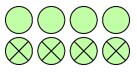Sol.10. No. of circles having X in them = 4
Total no. of circles = 8
Fraction of circles having X in them =$\cfrac { 4 }{ 8 }$ =$\cfrac { 1 }{ 2 }$

Ques. 11. Kristin received a CD player for her birthday. She bought 3 CDs and received 5 others as gifts. What fraction of her total CDs did she buy and what fraction did she receive as gifts?

Sol.11. No. of CDs bought = 3
No. of CDs gifted = 5
Total no. of CDs = 8
Fraction of CDs bought =$\cfrac { 3 }{ 8 }$
Fraction of CDs gifted =$\cfrac { 5 }{ 8 }$

___________________________________________________________________________

####NCERT Solutions Class 6

This Chapter contains the Exercises relating to the following topics , which are discussed in Chapter 7 – Fractions Class 6 NCERT book  : –

• 7.1 Introduction
• 7.2 A Fraction
• 7.3 Fraction on the Number Line
• 7.4 Proper Fractions
• 7.5 Improper and Mixed Fractions
• 7.6 Equivalent Fractions
• 7.7 Simplest Form of a Fraction
• 7.8 Like Fractions
• 7.9 Comparing Fractions
• 7.9.1 Comparing like fractions
• 7.9.2 Comparing unlike fractions
• 7.10 Addition and Subtraction of Fractions
• 7.10.1 Adding or subtracting like fractions
• 7.10.2 Adding and subtracting fractions